# [R Course] Data Visualization with R

Learn how to use the ggplot2 package to create visuals in R.

08-05-2019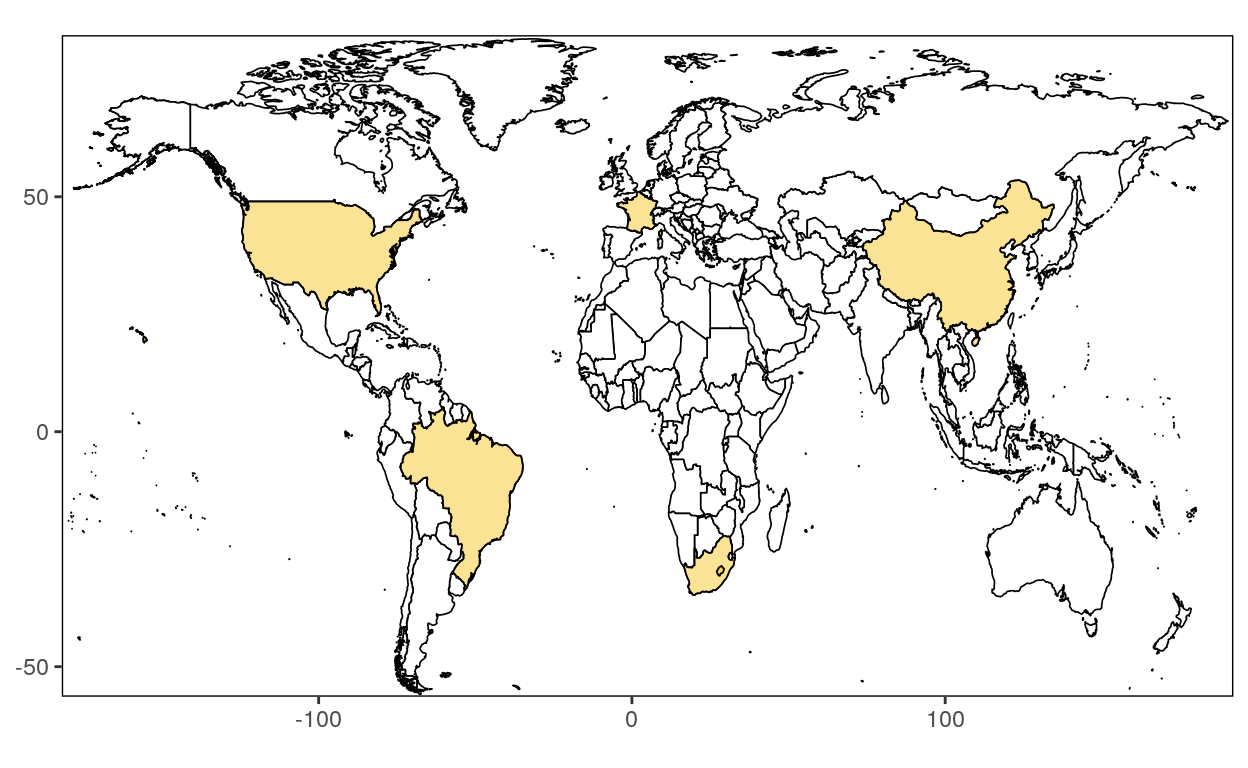## Set up

### Data

In this course, we will work with data stored in a Gsheet. Use the following code to load them!

``````library(gsheet)

``````
date country GDP section
2010 australia 1142250506 a
2011 australia 1389919156 d
2012 australia 1537477830 c
2013 australia 1563950959 e
2014 australia 1454675480 e
2015 australia 1339539063 f
2010 belgium 483577483 c
2011 belgium 526975257 e
2012 belgium 497815990 a
2013 belgium 521370528 f
2014 belgium 531234804 d
2015 belgium 454039037 d

### Package

For the examples to work, we need to load the `ggplot2` package.

``````library(ggplot2)
``````

## Ggplot

### Create a new ggplot

`ggplot()` initializes a ggplot object. It can be used to declare the input data frame for a graphic and to specify the set of plot aesthetics intended to be common throughout all subsequent layers unless specifically overridden.

``````ggplot(data = NULL, mapping = aes(), ...,)
``````

`ggplot()` alone do not work but is necessary to start a new plot. We need to add functions to produce, bar charts, line charts, etc.

The sections above will teach you how to add these functions.

## Bar Chart

There are two functions to create bar charts: geom_bar() and geom_col().

### Geom_bar

`geom_bar()` makes the height of the bar proportional to the number of cases in each group (or if the weight aesthetic is supplied, the sum of the weights). If you want the heights of the bars to represent values in the data, use geom_col() instead. geom_bar() uses stat_count() by default: it counts the number of cases at each x position.

``````geom_bar(mapping = NULL, data = NULL, stat = "count", position = "stack", ..., width = NULL,
binwidth = NULL, na.rm = FALSE, show.legend = NA, inherit.aes = TRUE)
``````

#### Counts

Create bar charts that show counts (or sums of weights):

``````# Number of times each country is represented in the data frame
# It represents also the number of year for each country
ggplot(dataGraph, aes(country)) +
geom_bar()
``````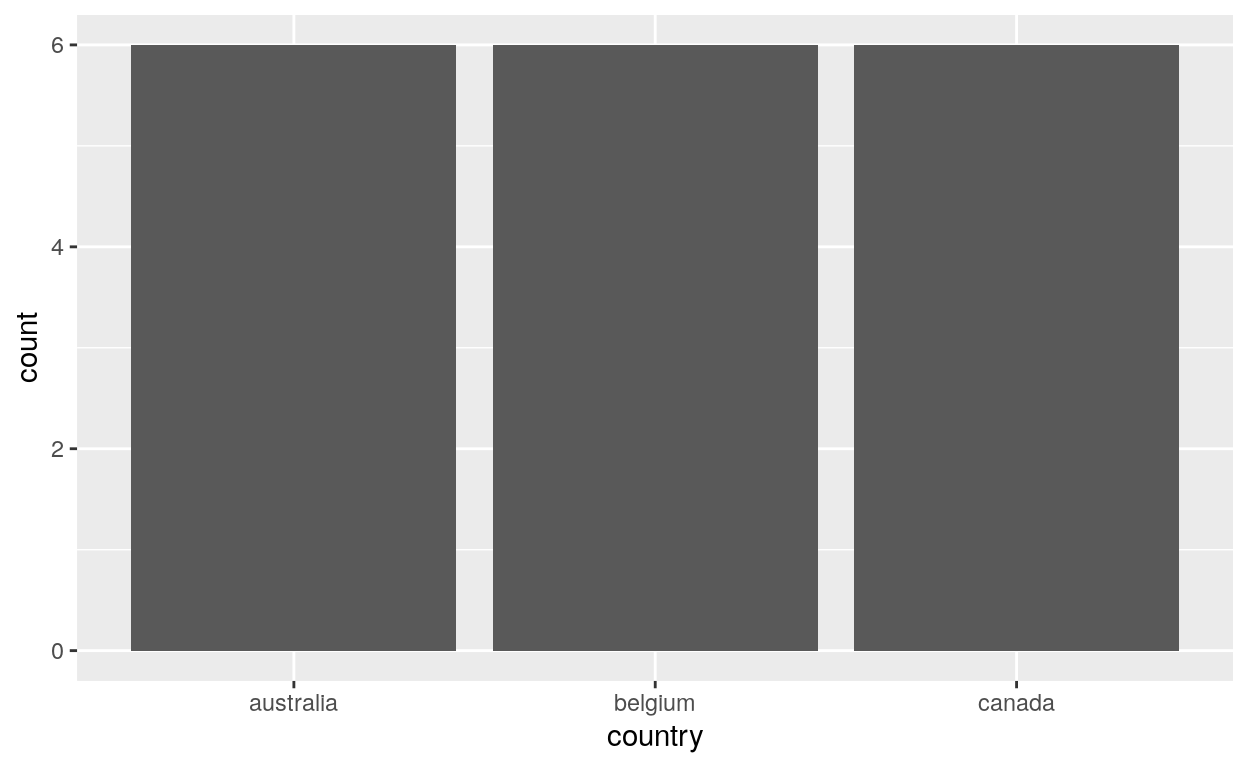#### Stat identity

Create bar charts that put columns for the x and y axis using the option stat identity:

``````ggplot(dataGraph, aes(x=country, y=GDP)) +
geom_bar(stat = "identity")
``````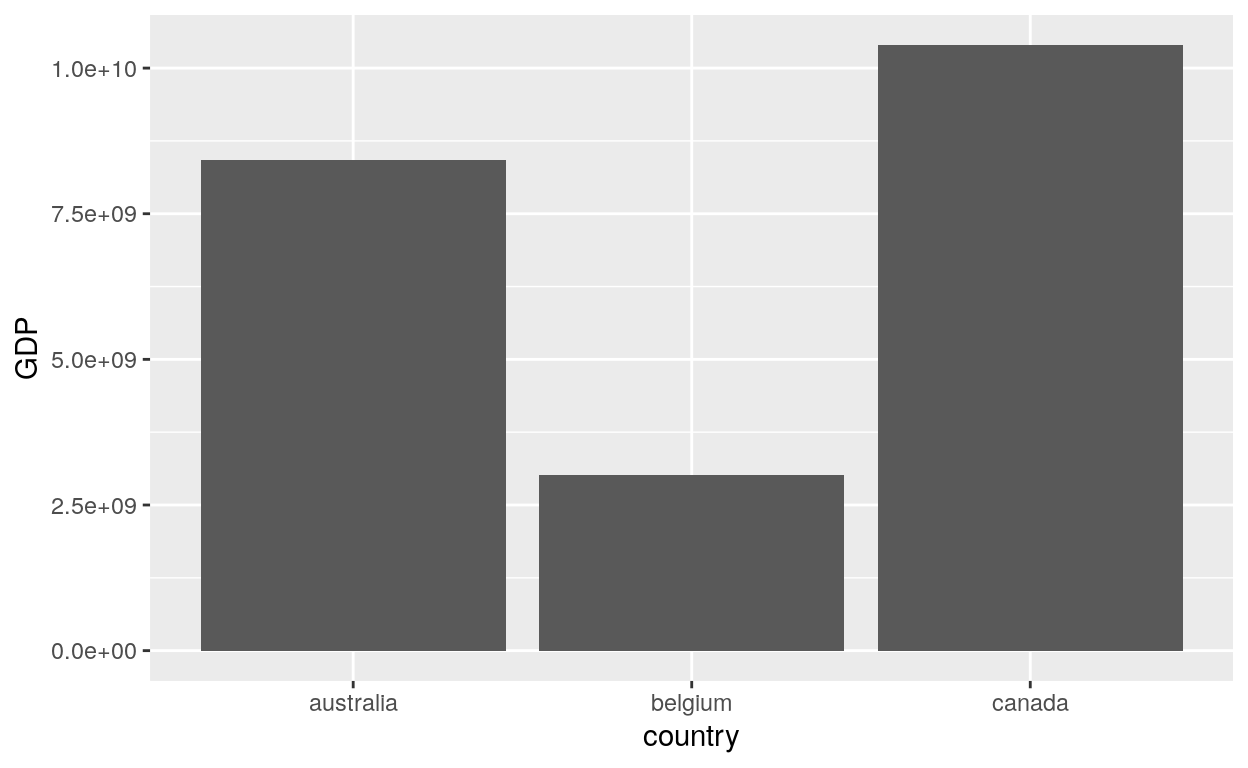It’s the option stat = “identity” that allow the function `geom_bar` to produce the y axis with the GDP as the value. Without this option, you will receive an error!

#### Fill

Create bar charts with the fill option:

``````# Bar charts are automatically stacked when multiple bars are placed at the same location.
# The order of the fill in the graph is designed to match the legend
# We see here that each color correspond to each year
ggplot(dataGraph, aes(x=country, y=GDP, fill = date)) +
geom_bar(stat = "identity")
``````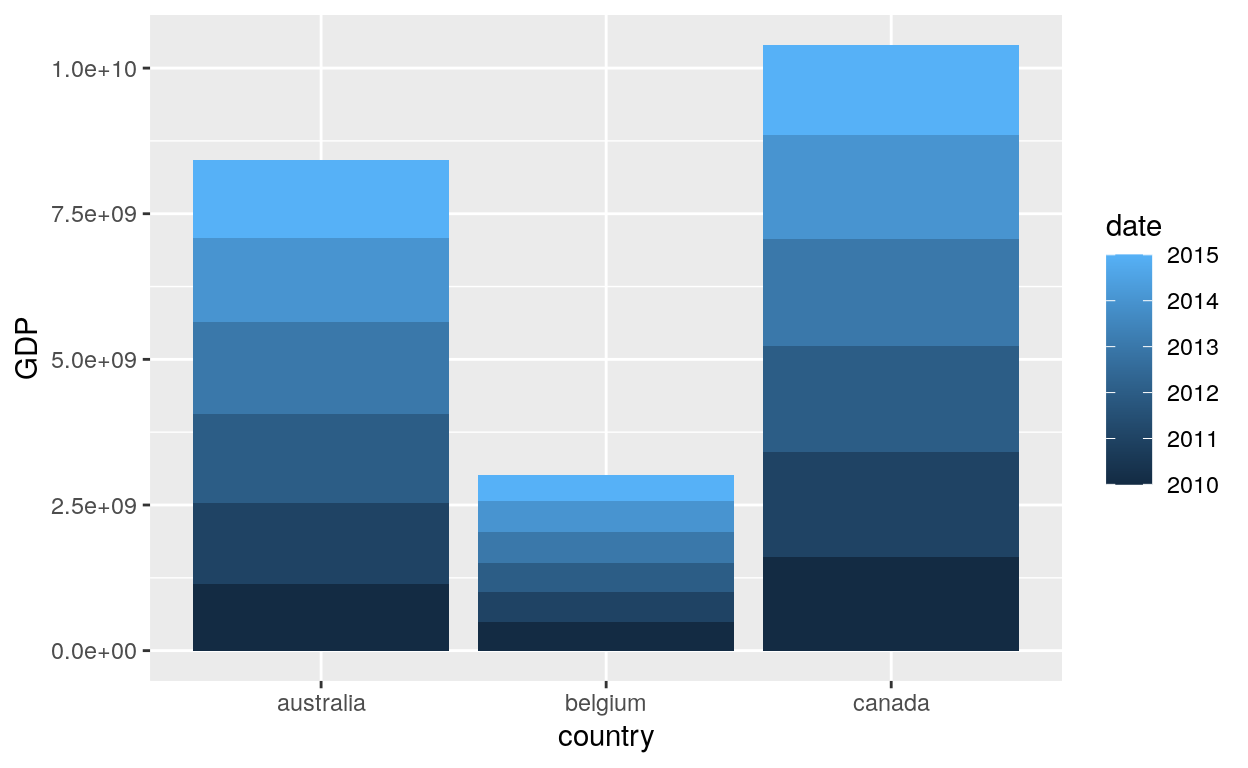#### Dodge2

Create bar charts with the dodge2 option:

``````# Adding:
# position = "dodge2": To put each year as separeted column
ggplot(dataGraph, aes(x=country, y=GDP, fill = date)) +
geom_bar(stat = "identity", position = "dodge2")
``````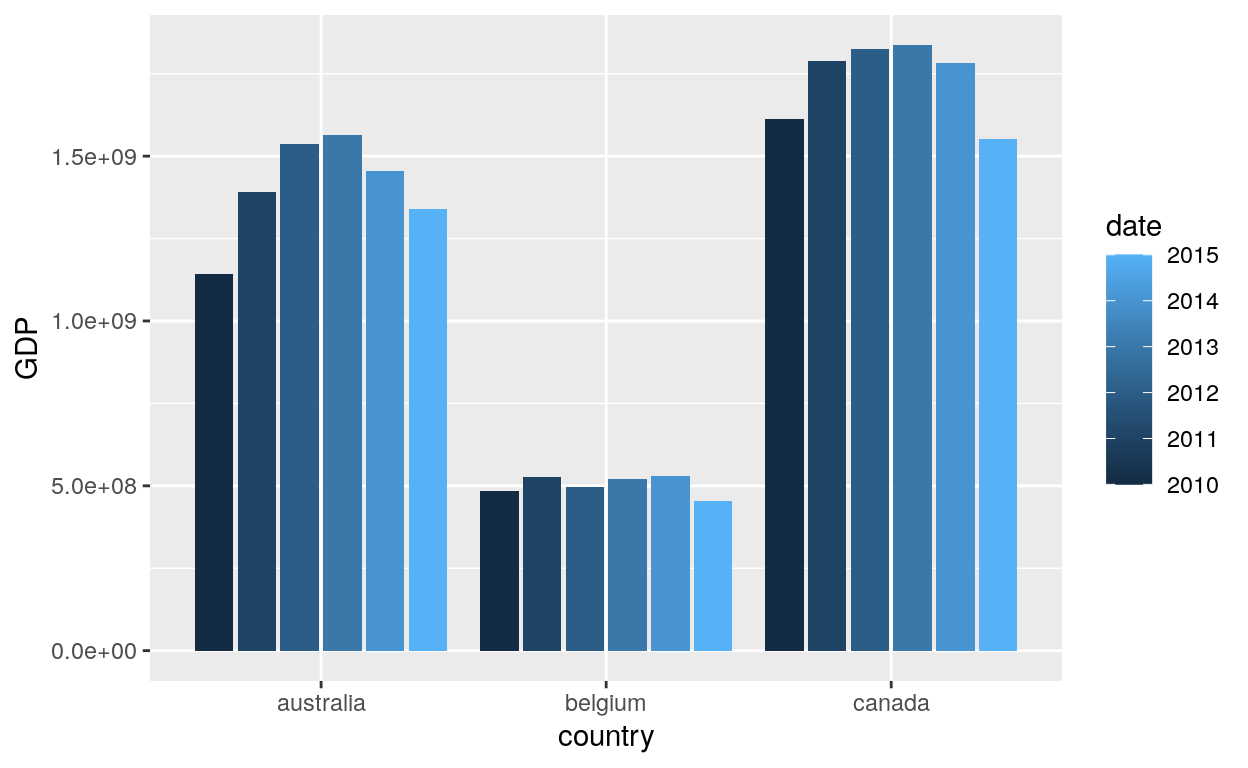#### Dodge and Width

Create bar charts with a width option:

``````# date as the x axis
# GDP as the y axis
# country as fill
# position = dodge
# the width of the bars as 0.5
ggplot(data = dataGraph, aes(x = date, y = GDP, fill = country)) +
geom_bar(stat = "identity", width = 0.5, position = "dodge")
``````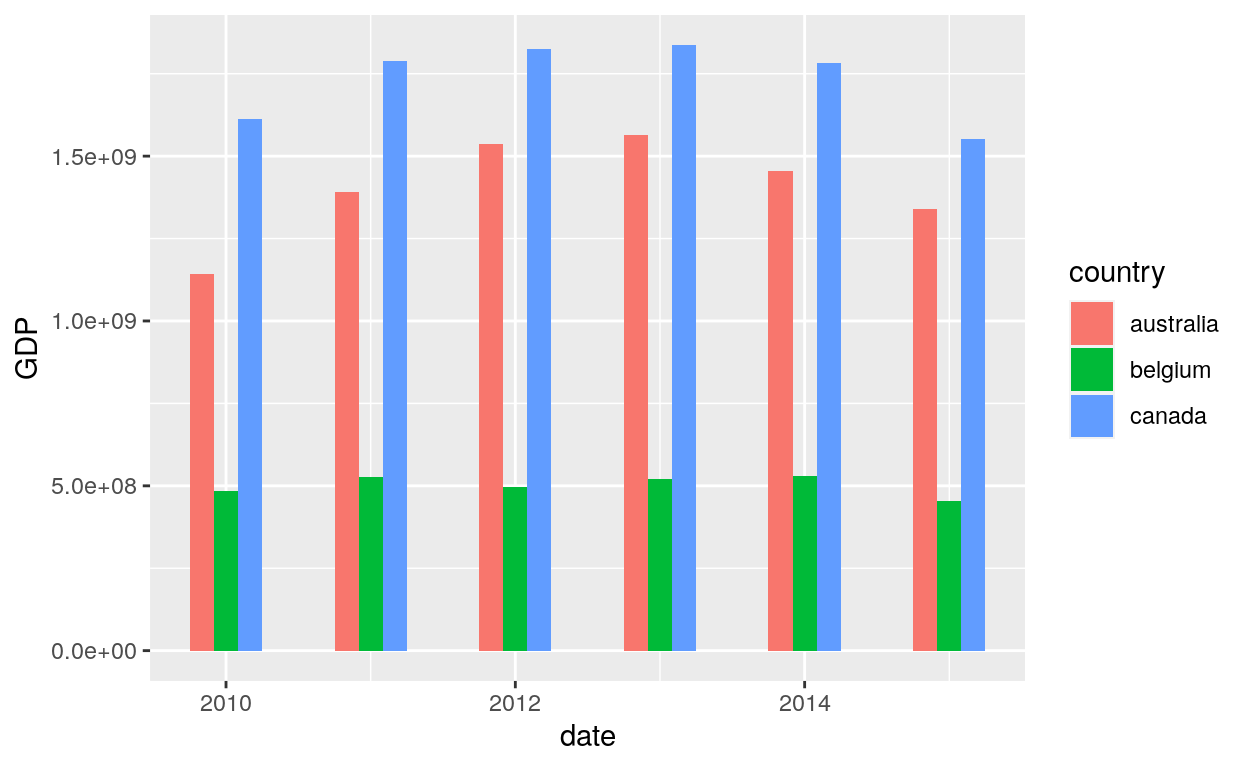### Geom_col

`geom_col()` uses the option stat_identity by default to leave the data as is.

``````geom_col(mapping = NULL, data = NULL, position = "stack", ..., width = NULL,
na.rm = FALSE, show.legend = NA, inherit.aes = TRUE)
``````

#### Bars

``````# Using geom_col with:
# Country as x axis
# GDP as y axis
ggplot(data = dataGraph, aes(x = country, y = GDP)) +
geom_col()
``````#### Width

``````# Adding:
# the fill option:  meaning that each bar (each country) has a different colour
# the width option: change the bar size
ggplot(data = dataGraph, aes(x = country, y = GDP, fill = country)) +
geom_col(width = 0.8)
``````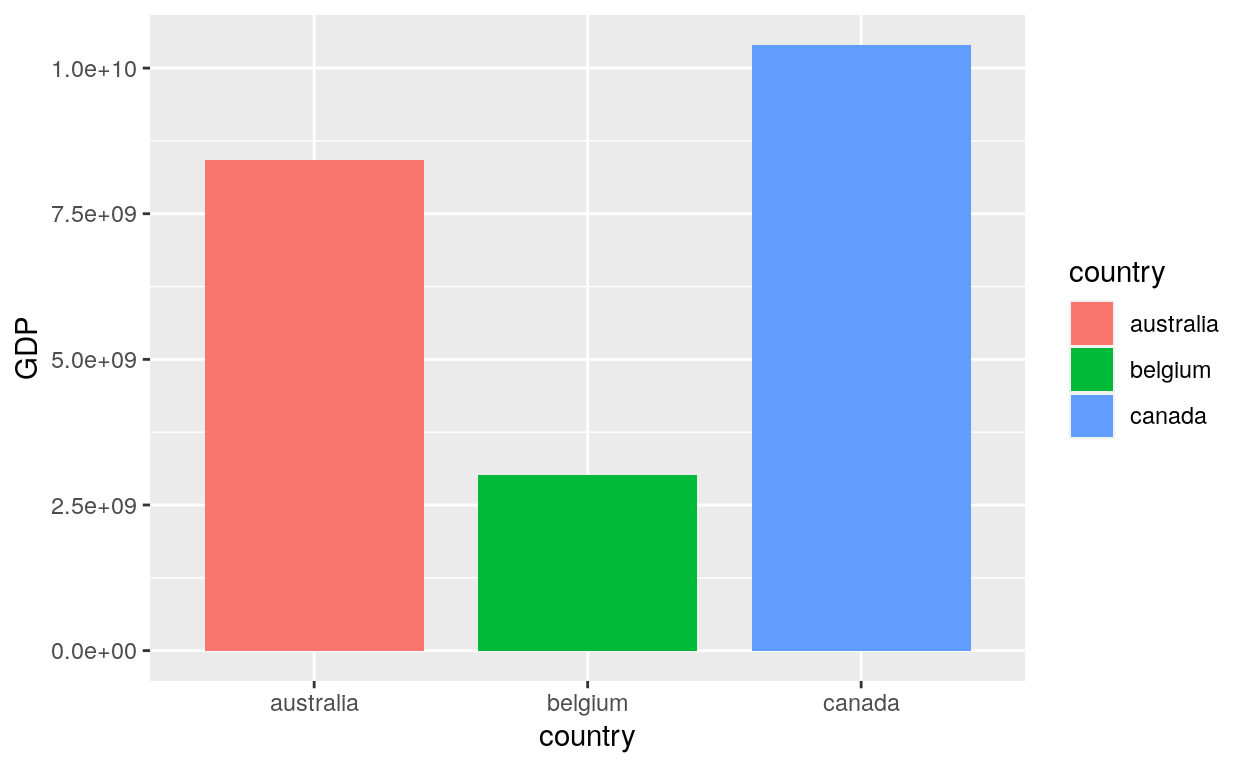## Line Chart

### Geom_line

`geom_line()` connects them in order of the variable on the x axis.

``````geom_line(mapping = NULL, data = NULL, stat = "identity",
position = "identity", na.rm = FALSE, show.legend = NA,
inherit.aes = TRUE, ...)
``````

#### Multiple Lines

`geom_line()` is suitable for time series:

``````ggplot(data = dataGraph, aes(x = date, y = GDP, colour = country)) +
geom_line()
``````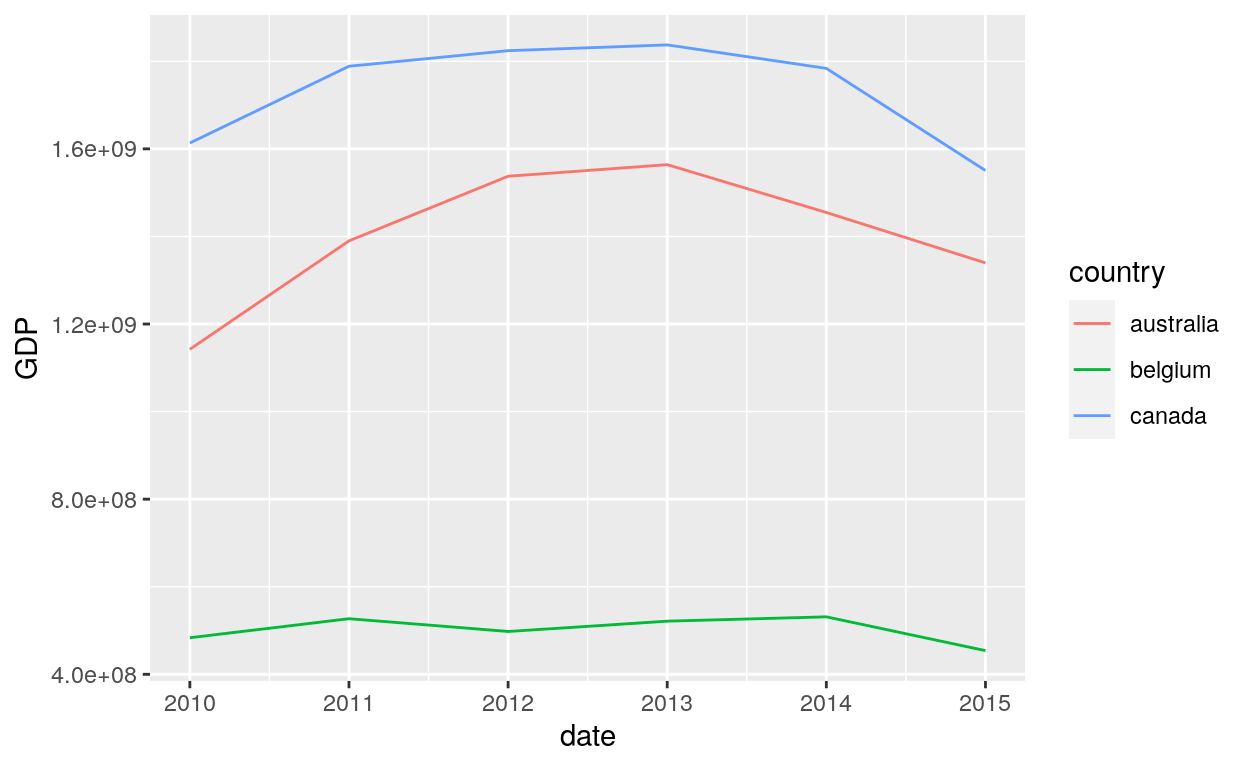#### Unique line

If you wish to show the data for the Canada only:

``````# Filter to keep the country Canada
library(dplyr)
``````
``````ggplot(data = dataGraphCanada, aes(x = date, y = GDP)) +
geom_line()
``````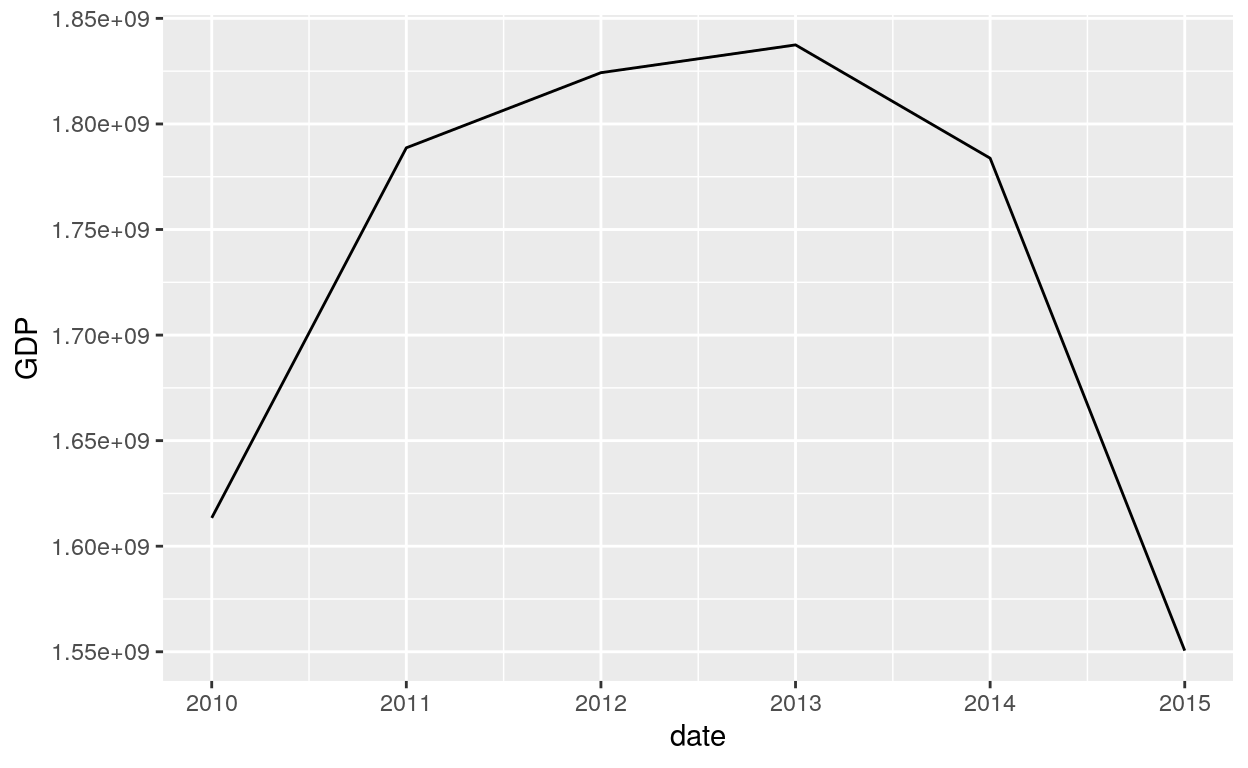#### Aes Colour

``````ggplot(data = dataGraphCanada, aes(x = date, y = GDP, colour = date)) +
geom_line()
``````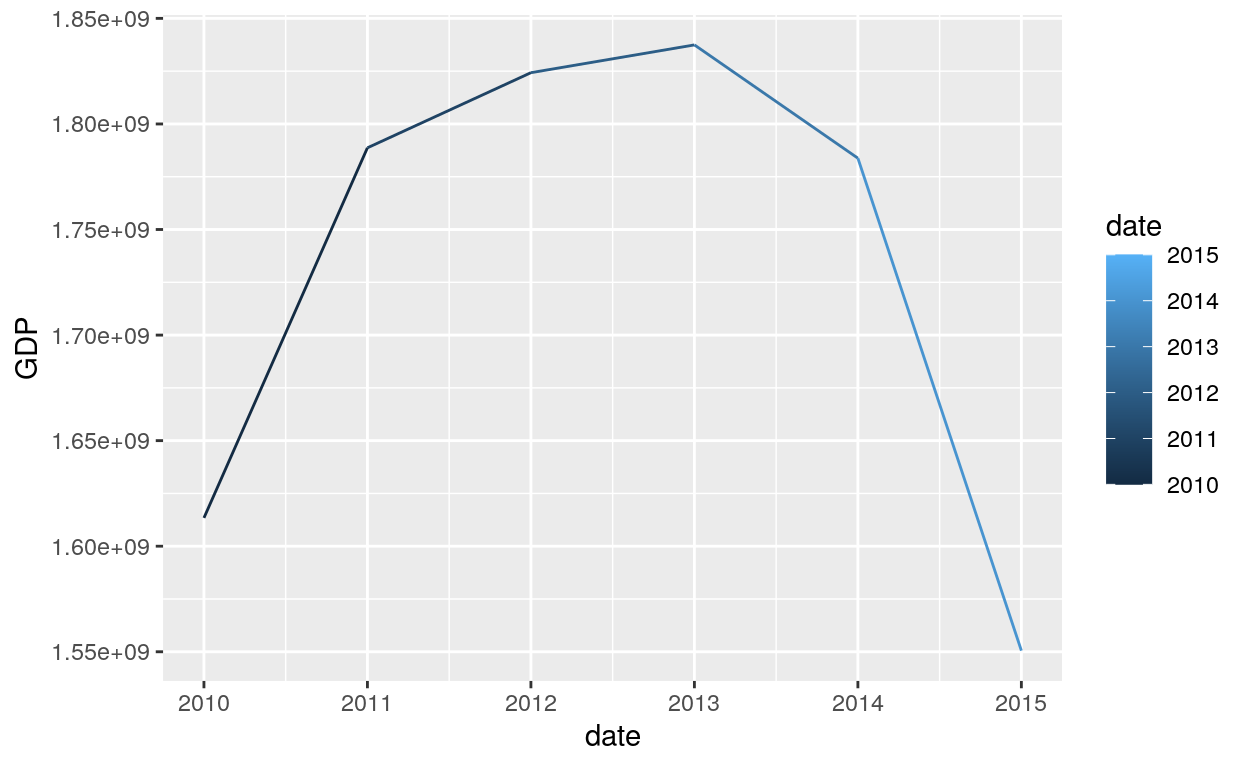#### Colour

``````# Changing the colour parameter to red
geom_line(colour = "red")
``````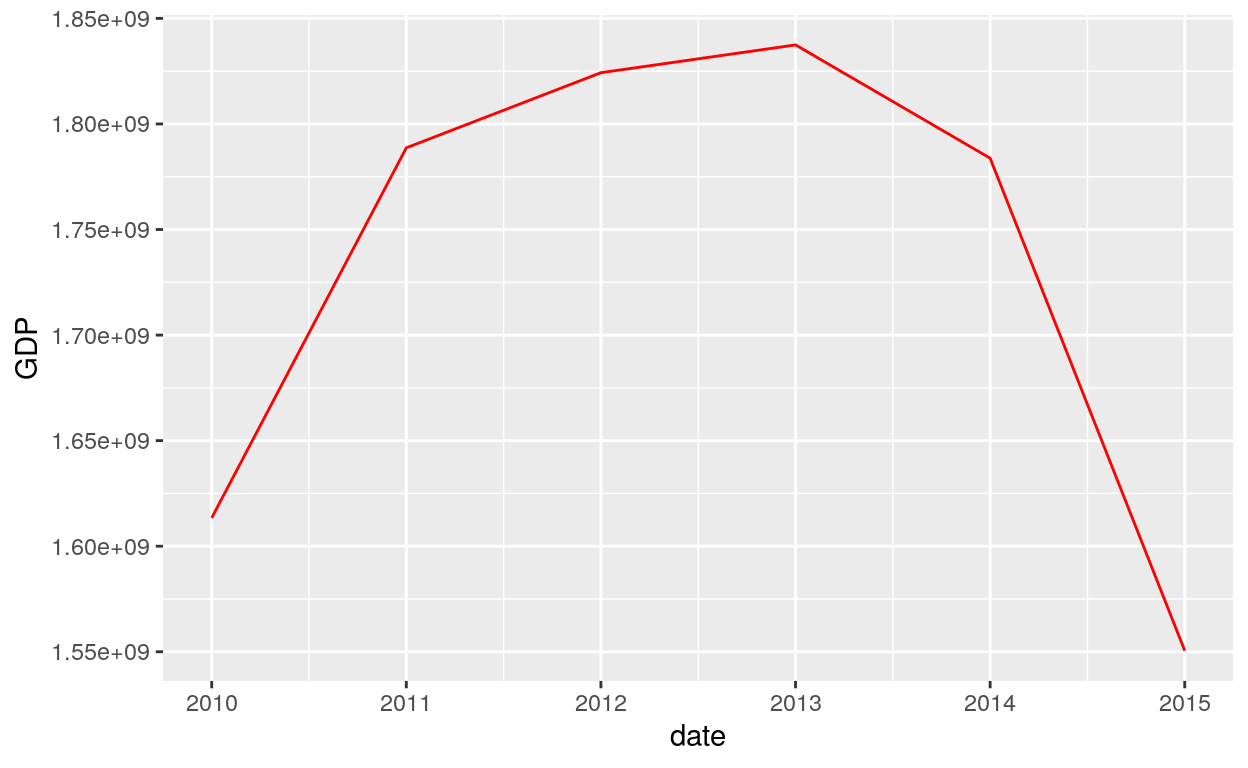#### Arrow

``````# Use the arrow parameter to add an arrow to the line
geom_line(colour = "red", arrow = arrow())
``````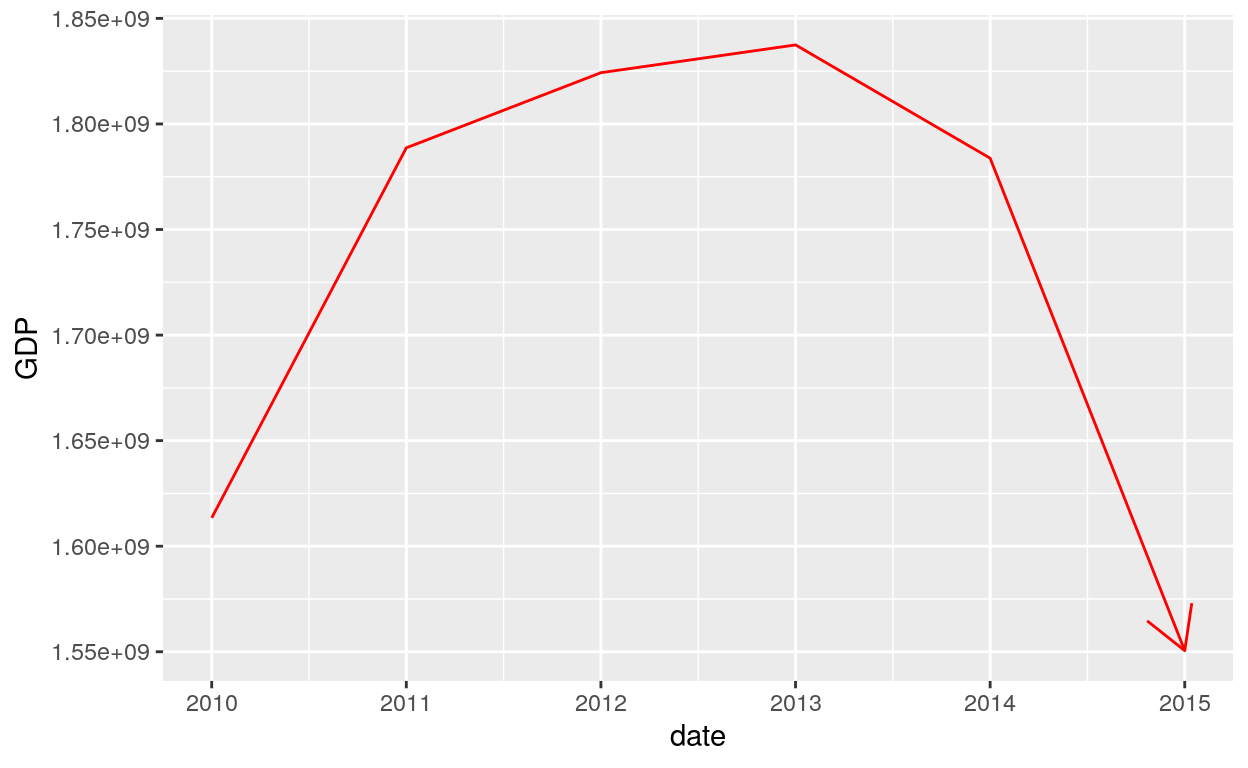``````ggplot(dataGraphCanada, aes(date, GDP)) +
geom_line(colour = "blue", arrow = arrow(angle = 15, ends = "both", type = "closed"))
``````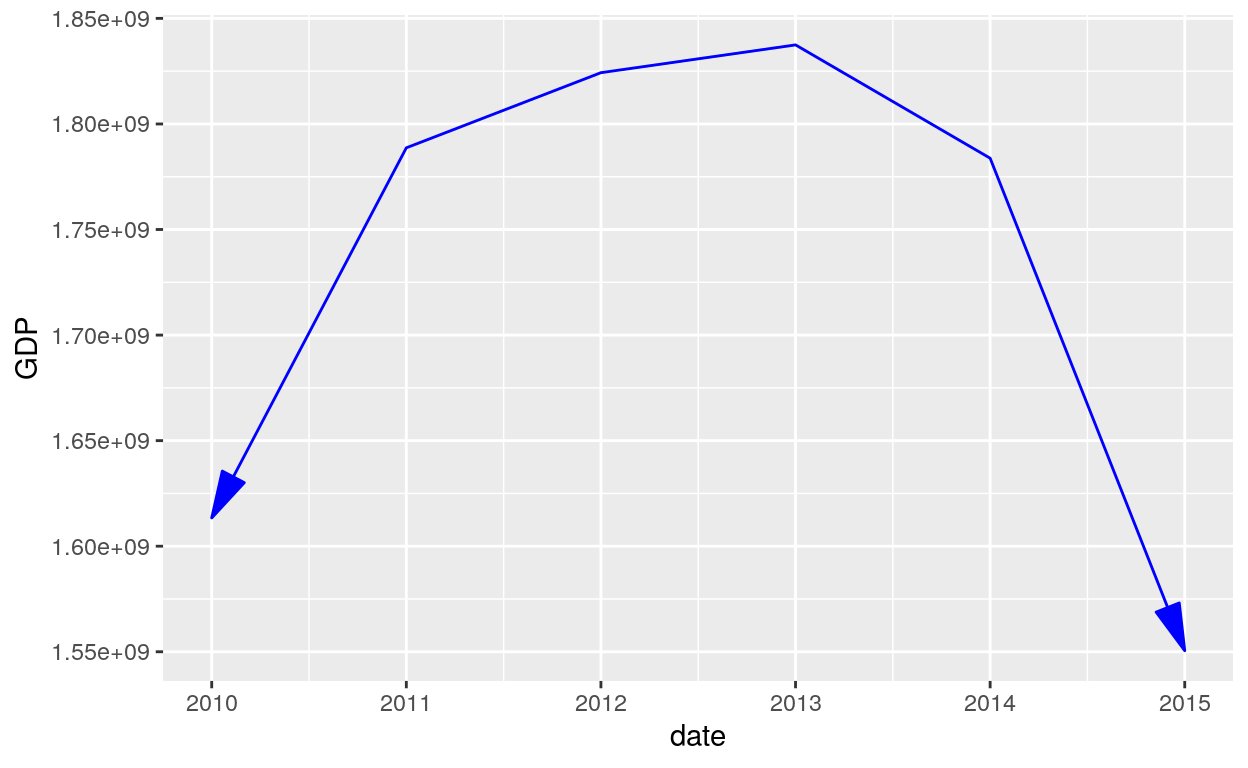#### Linetype

``````# Setting line type
geom_line(colour = "blue", linetype = 2, arrow = arrow(angle = 30, type = "closed"))
``````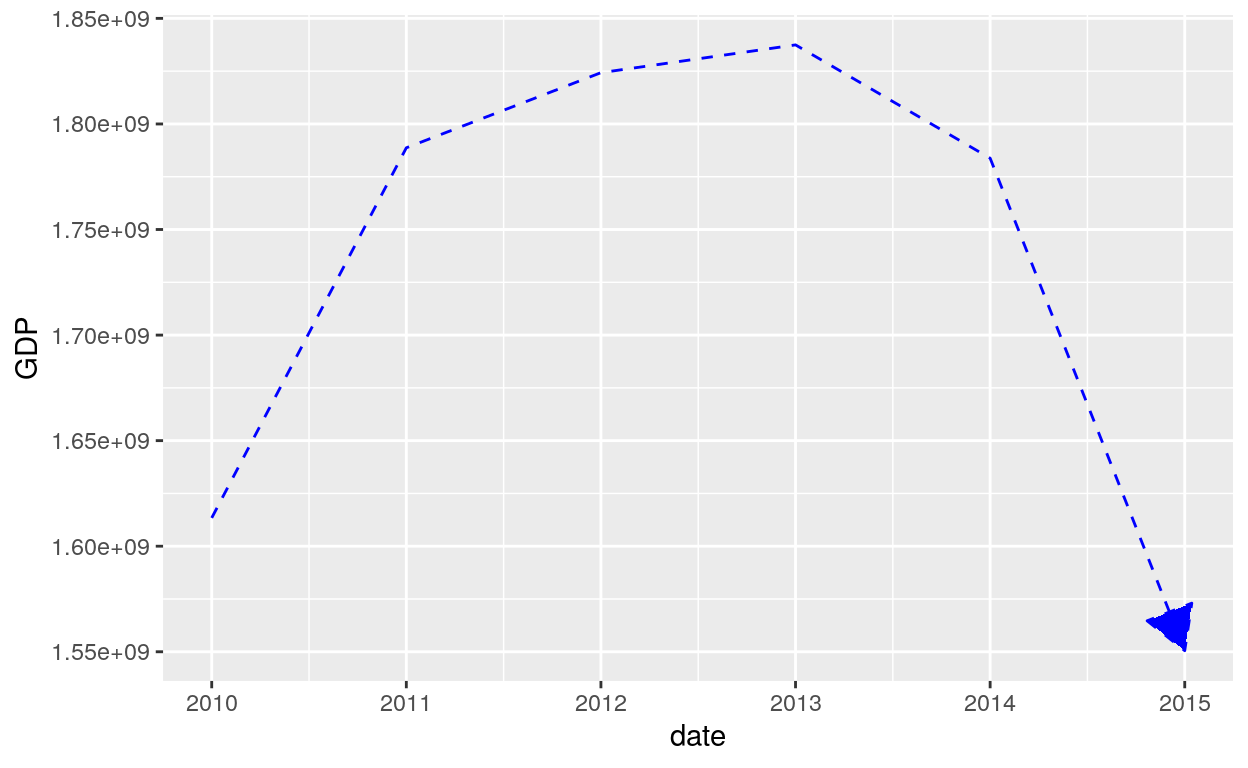``````# Setting line type
geom_line(colour = "purple", linetype = 4, arrow = arrow())
``````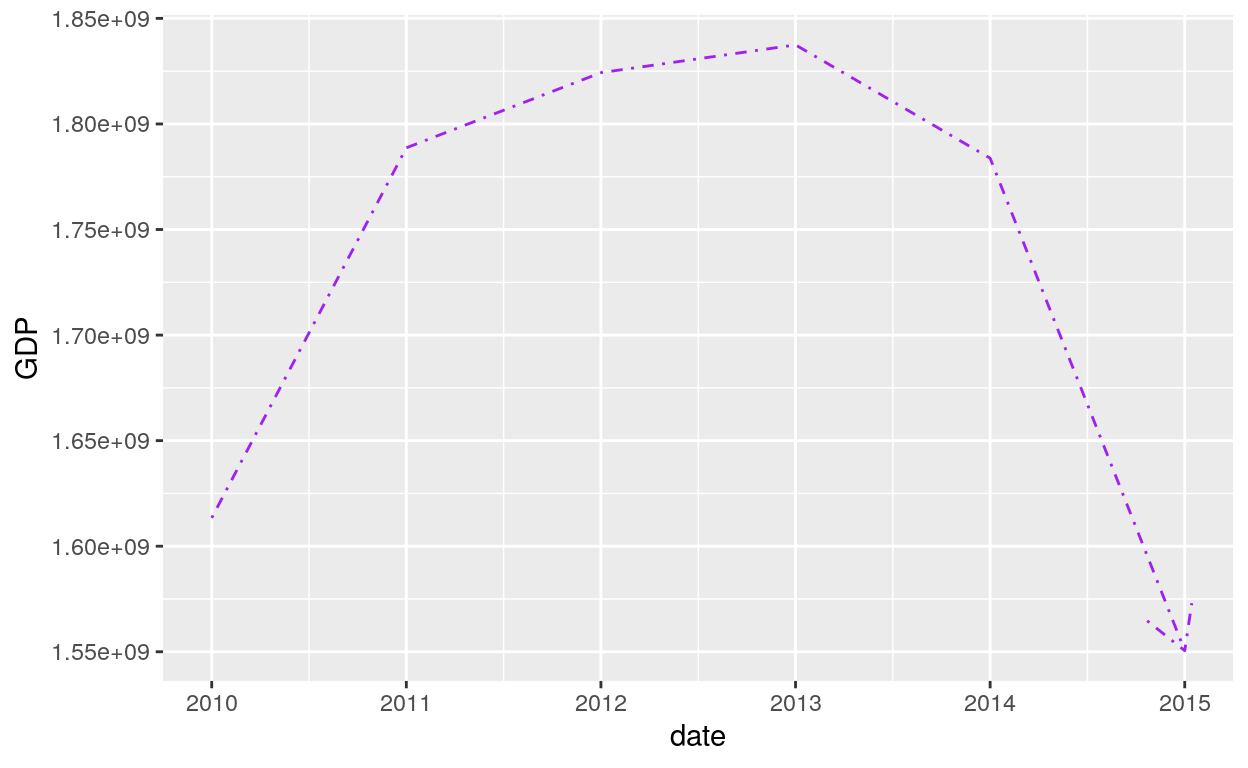### Geom_step

`geom_step()` creates a stairstep plot, highlighting exactly when changes occur. The group aesthetic determines which cases are connected together.

``````geom_step(mapping = NULL, data = NULL, stat = "identity",
position = "identity", direction = "hv", na.rm = FALSE,
show.legend = NA, inherit.aes = TRUE, ...)
``````

#### Steps

`geom_step()` is useful when you want to highlight exactly when the y value changes:

``````ggplot(data = dataGraphCanada, aes(x = date, y = GDP)) +
geom_step()
``````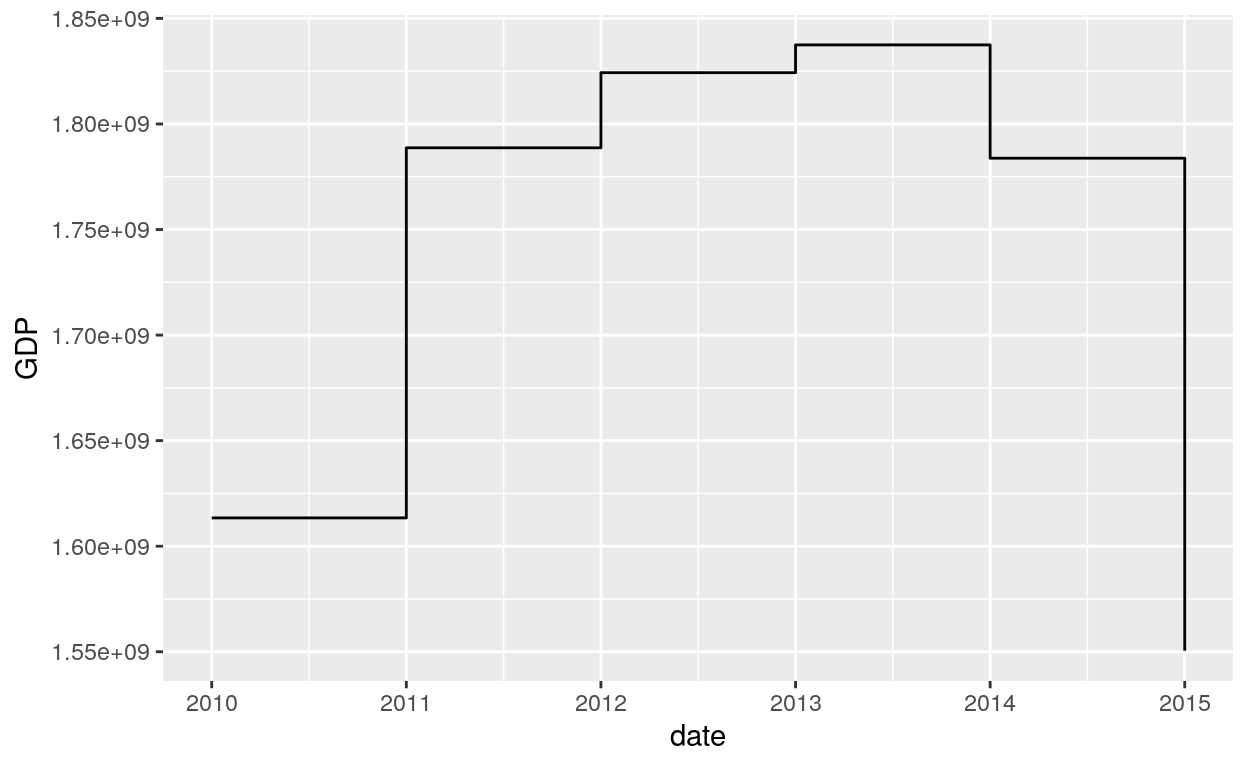## Scatterplot

### Geom_point

The `geom_point()` is used to create scatterplots. The scatterplot is most useful for displaying the relationship between two continuous variables. It can be used to compare one continuous and one categorical variable, or two categorical variables, but a variation like geom_jitter(), geom_count(), or geom_bin2d() is usually more appropriate. A bubblechart is a scatterplot with a third variable mapped to the size of points.

``````geom_point(mapping = NULL, data = NULL, stat = "identity", position = "identity", ...,
na.rm = FALSE, show.legend = NA, inherit.aes = TRUE)
``````

#### Points

``````ggplot(dataGraph, aes(country, GDP)) +
geom_point()
``````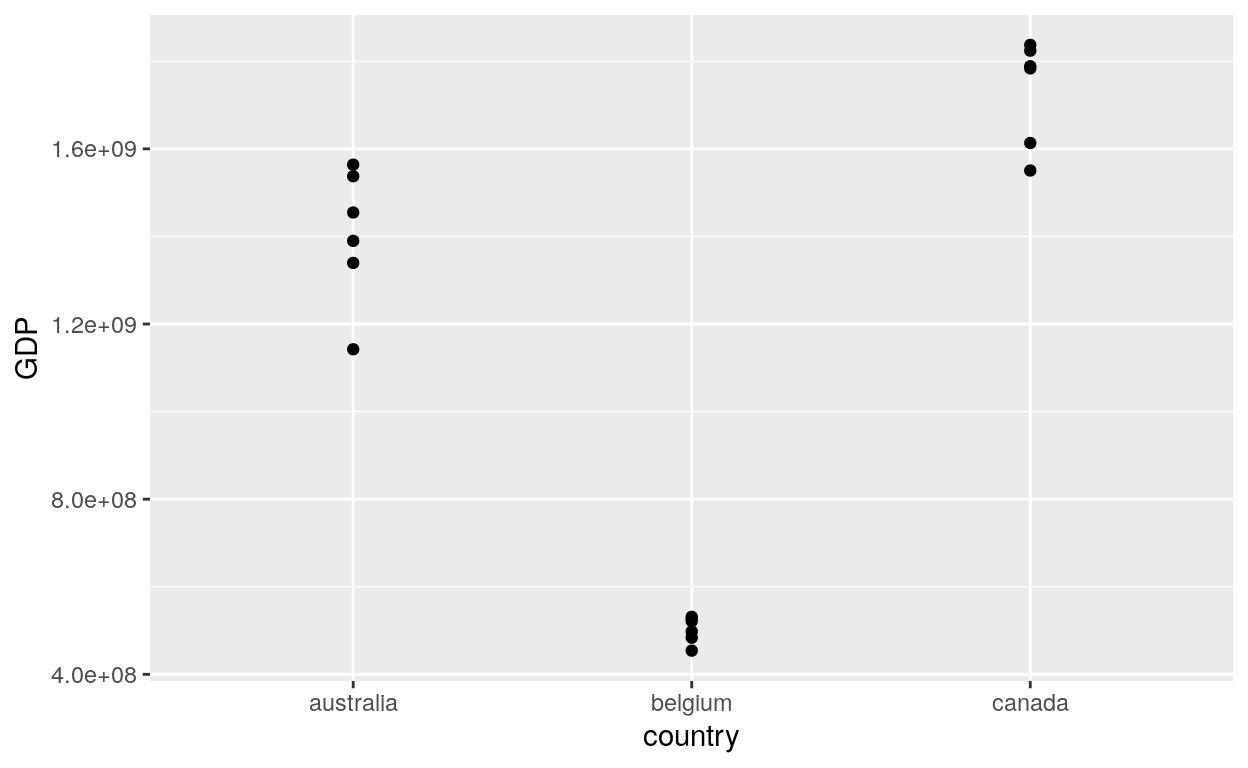#### Aes Colour

``````# Add aesthetic mappings
ggplot(dataGraph, aes(country, GDP, colour = country)) +
geom_point()
``````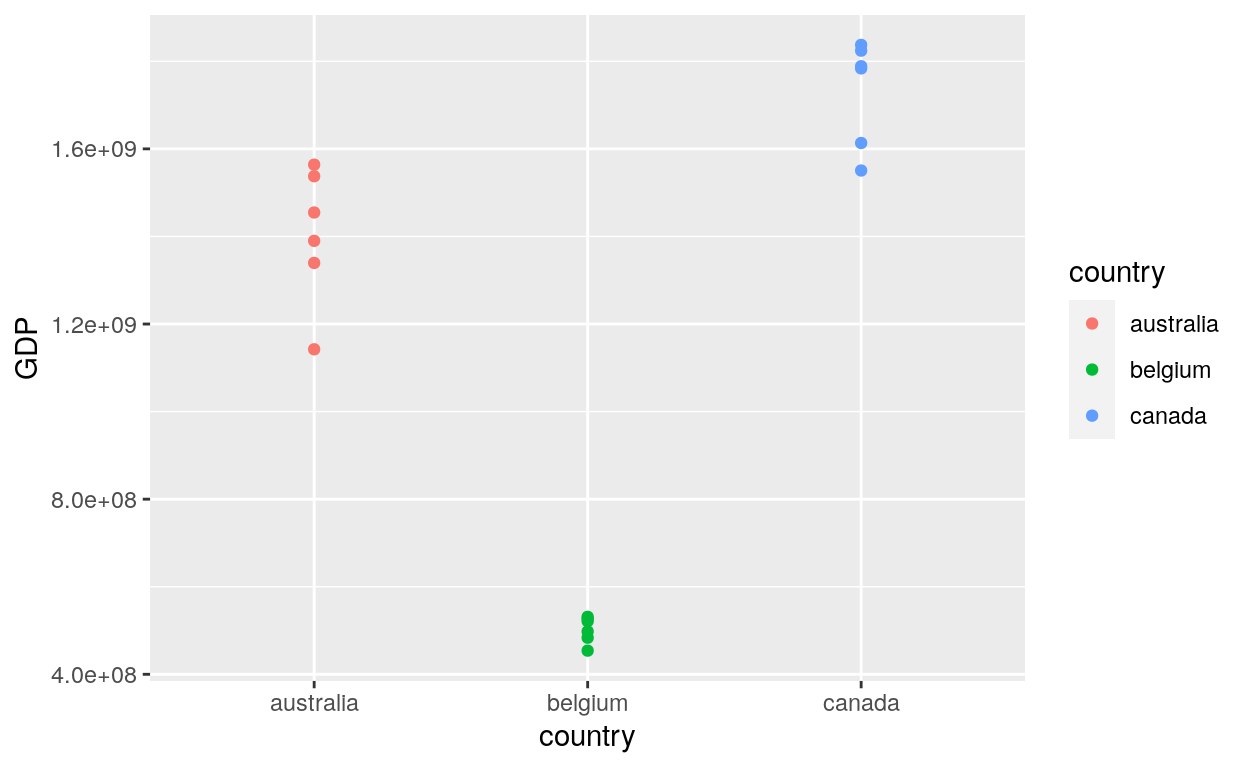#### Aes Size

Set points size by using the option size in the `aes()` function:

``````ggplot(dataGraph, aes(country, GDP, colour = country, size = GDP)) +
geom_point()
``````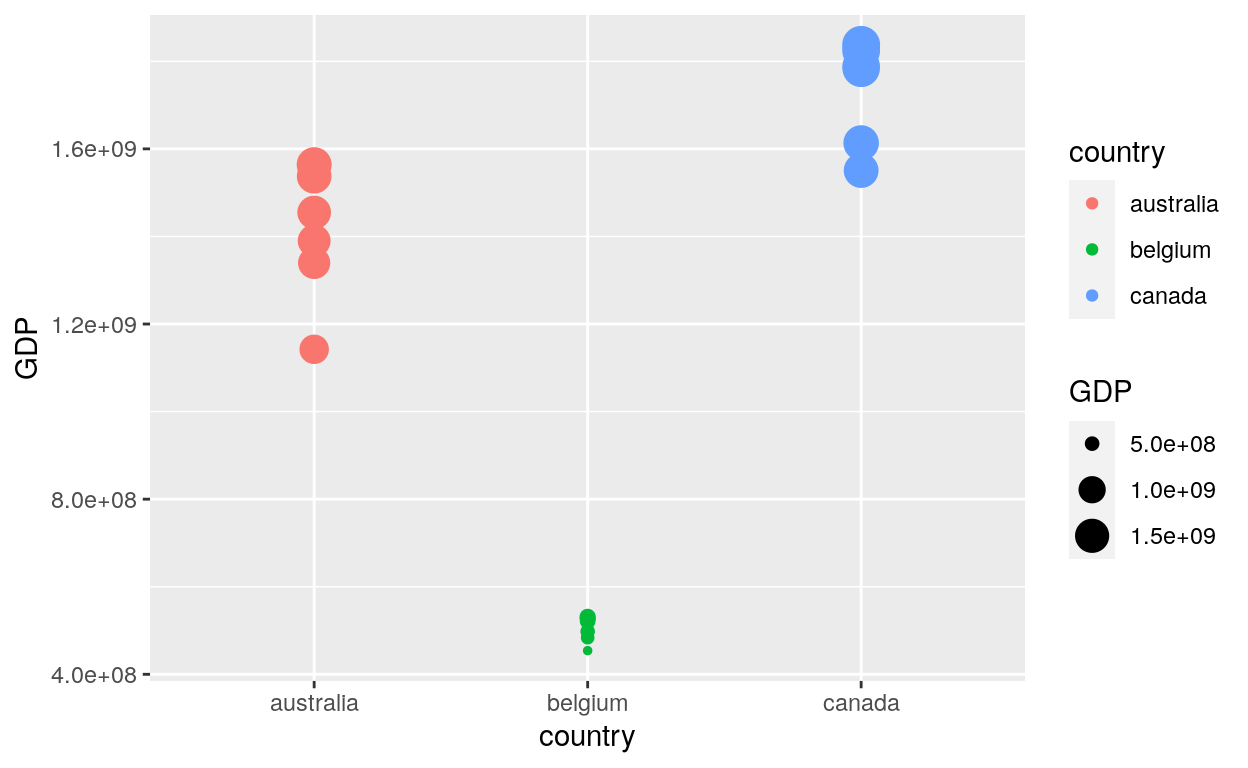#### Fixed colour and size

``````# Set aesthetics to fixed value
ggplot(dataGraph, aes(country, GDP)) +
geom_point(colour = "red", size = 3)
``````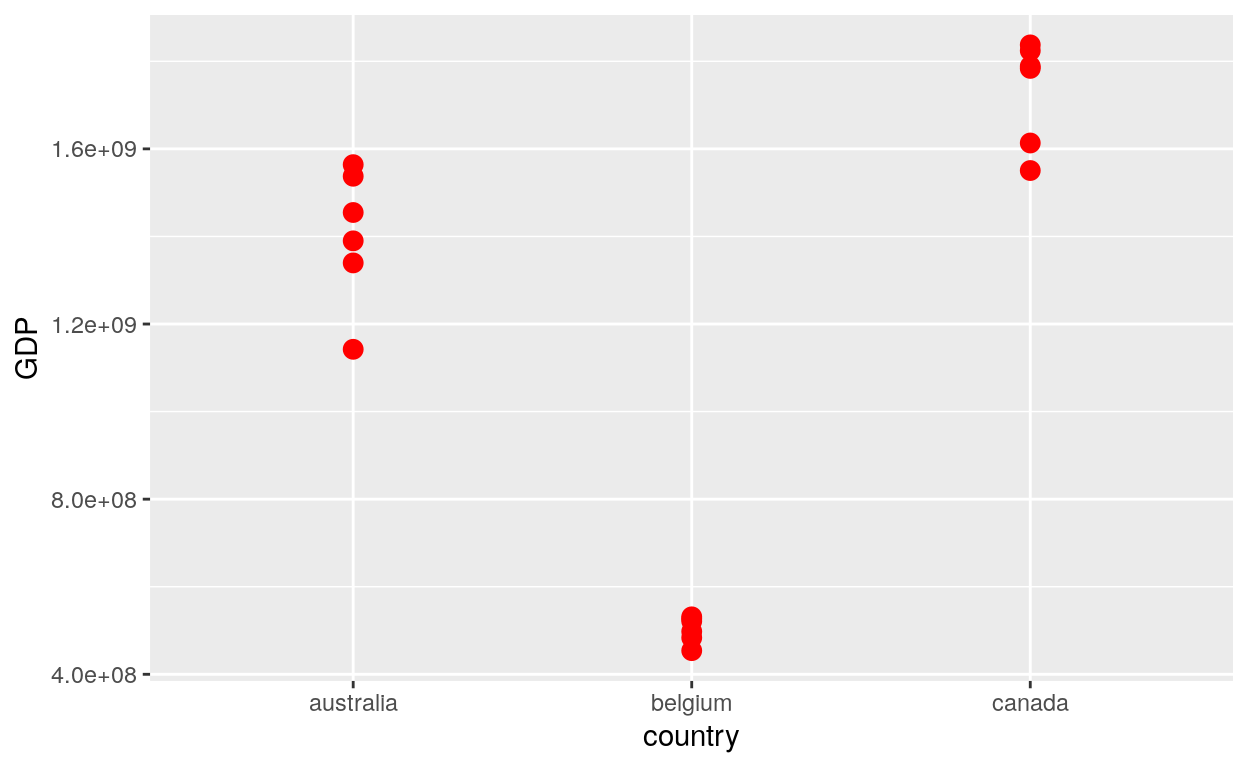#### Shape, Fill and Stroke

Set up the shape, fill and stroke

``````# For shapes that have a border (like 21), you can colour the inside and outside separately.
# Use the stroke aesthetic to modify the width of the border
ggplot(dataGraph, aes(country, GDP)) +
geom_point(shape = 21, colour = "black", fill = "white", size = 5, stroke = 5)
``````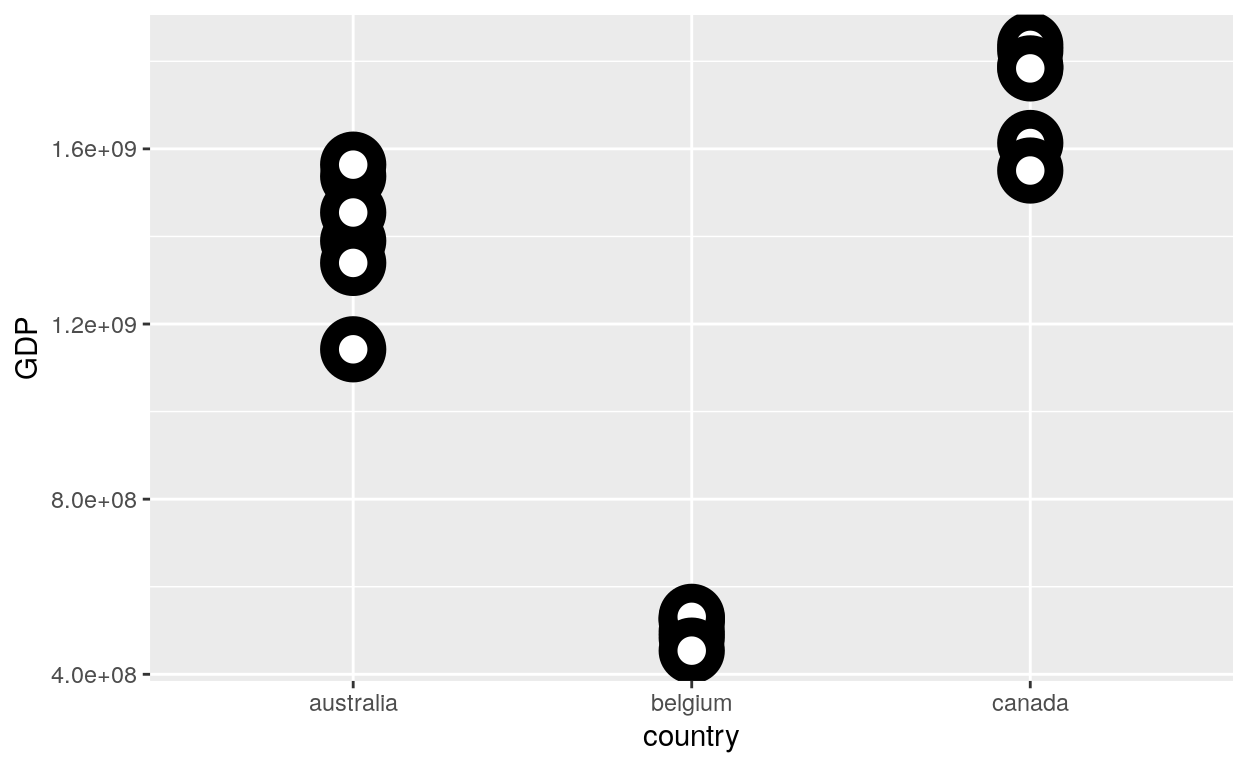#### Layering Points

``````# You can create interesting shapes by layering multiple points of different sizes
ggplot(dataGraph, aes(country, GDP, shape = country)) +
geom_point(aes(colour = country), size = 4) +
geom_point(colour = "grey90", size = 1.5)
``````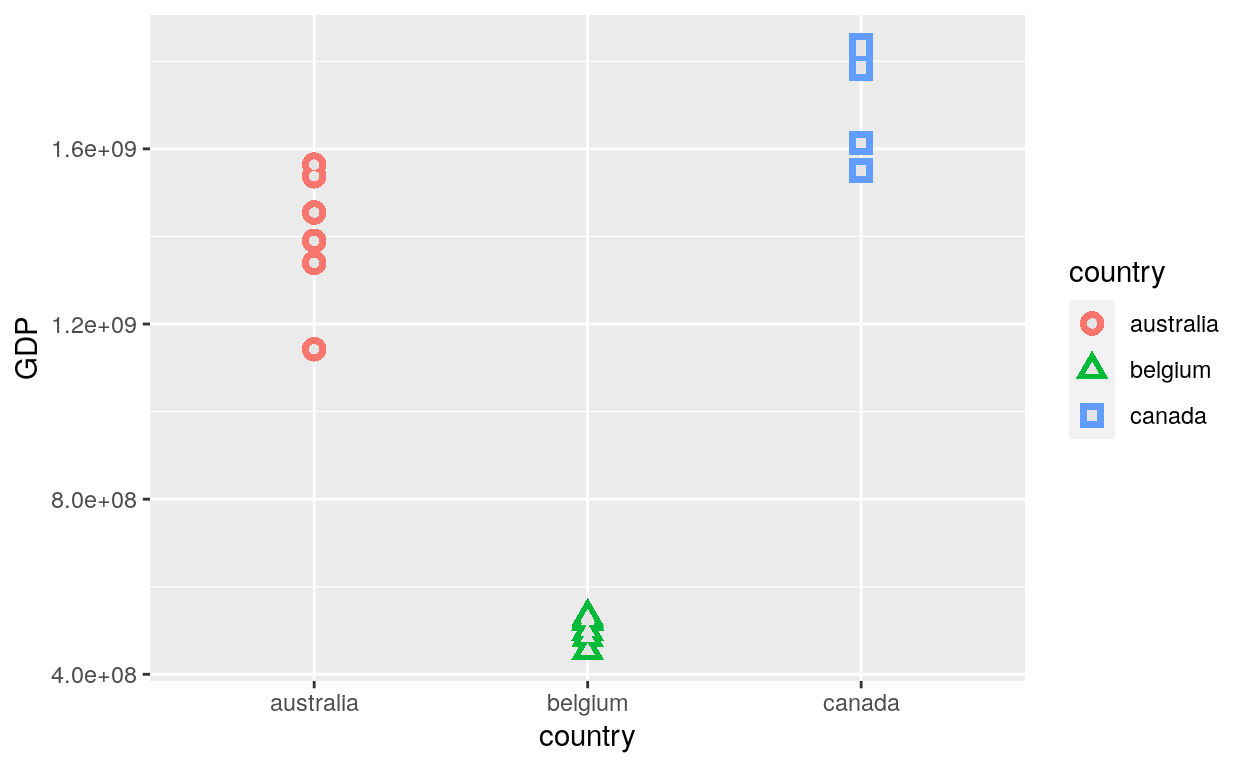``````ggplot(dataGraph, aes(country, GDP, shape = country)) +
geom_point(colour = "black", size = 4.5) +
geom_point(colour = "pink", size = 4) +
geom_point(aes(shape = factor(country)))
``````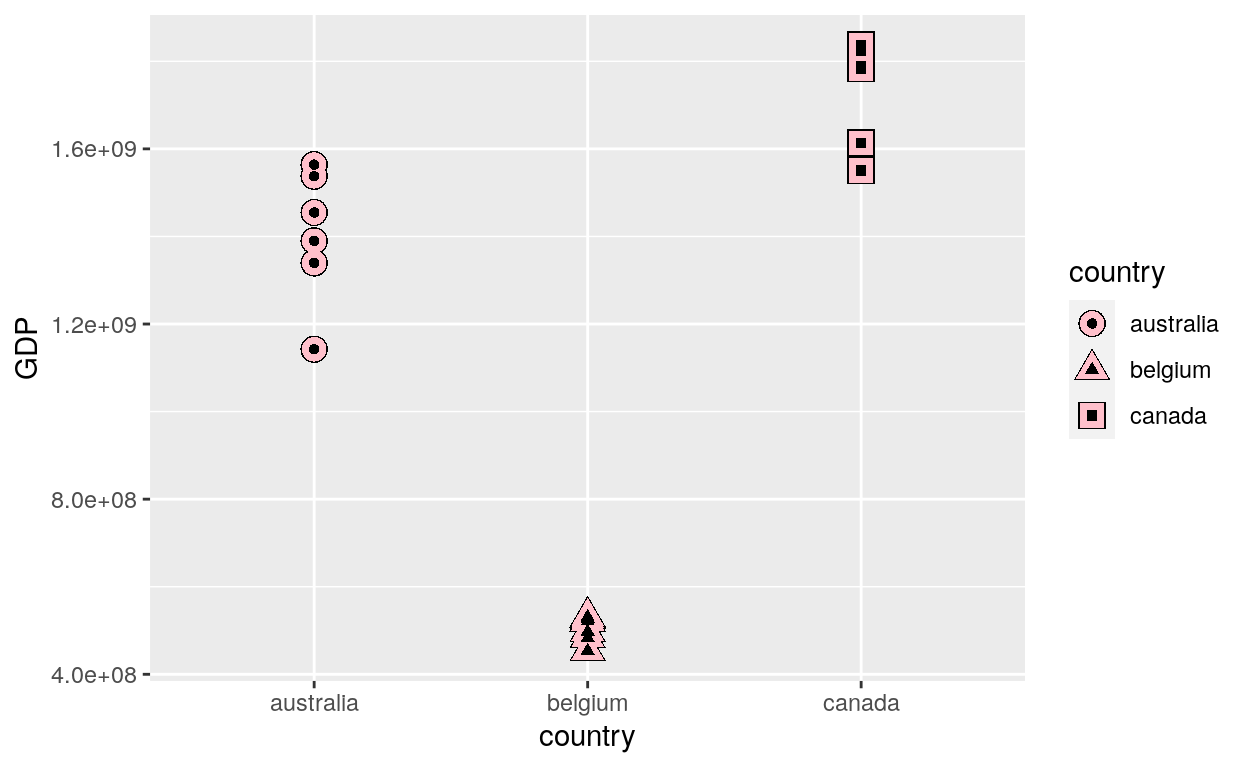#### Time series

The use of date as x axis for time series:

``````# geom_point() works for time series too
ggplot(data = dataGraph, aes(x = date, y = GDP, color = country)) +
geom_point(aes(size = GDP))
``````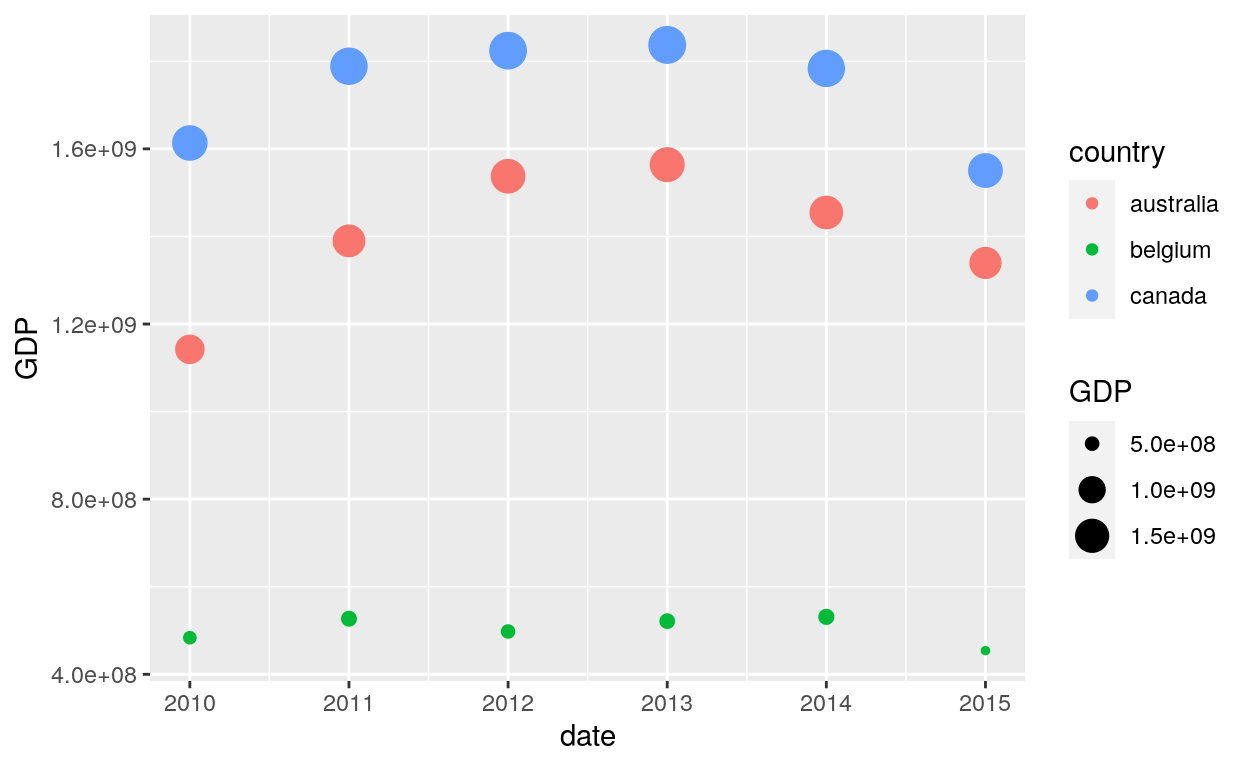#### Missing values

`Geom_point` is capable of handling missing value with the na.rm function:

``````# Create a column with random data from a sample of 1 to 500
dataGraph\$sample <- sample(1:500, 18, replace=F)
dataGraph <- transform(dataGraph, column.na = ifelse(sample(1:500, 18, replace=F) < 150, NA, sample))
``````

A warning message is displayed:

``````# geom_point warns when missing values have been dropped from the data set and not plotted
# you can turn this off by setting na.rm = TRUE
ggplot(dataGraph, aes(GDP, column.na)) +
geom_point()
``````
``Warning: Removed 5 rows containing missing values (geom_point).``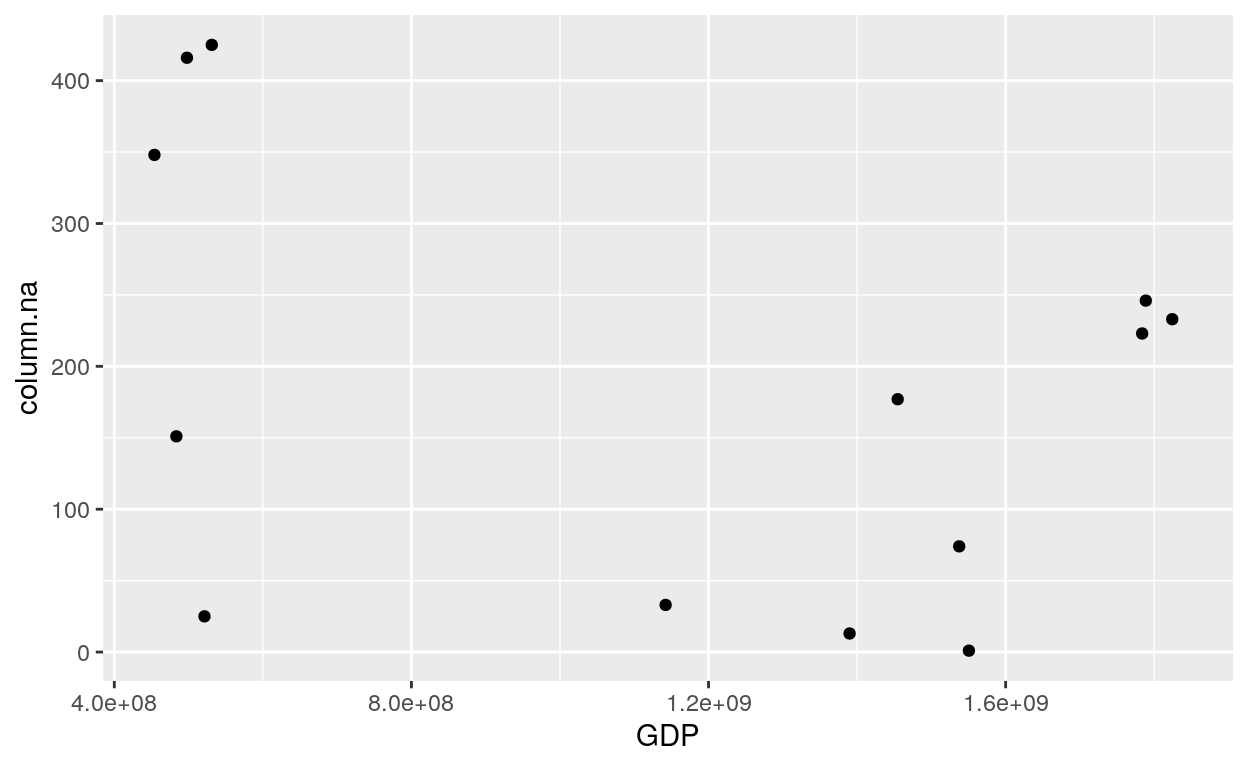There is no more warning message:

``````# Add TRUE to na.rm
ggplot(dataGraph, aes(GDP, column.na)) +
geom_point(na.rm = TRUE)
``````## Maps

This set of geom, stat, and coord are used to visualise simple feature (sf) objects. For simple plots, you will only need geom_sf() as it uses stat_sf() and adds coord_sf() for you. geom_sf() is an unusual geom because it will draw different geometric objects depending on what simple features are present in the data: you can get points, lines, or polygons.

### Geom_sf

``````geom_sf(mapping = aes(), data = NULL, stat = "sf", position = "identity",
na.rm = FALSE, show.legend = NA, inherit.aes = TRUE, ...)
``````

Setting up the data for the map:

``````# The package rnaturalearth provides data to create a map of the world.
# Use ne_countries to pull country data and choose the scale.
# The function ne_countries return sp classes by default.
# You can choose the sf classe, as defined in the argument returnclass.

library("sf")
library("rnaturalearth")

world <- ne_countries(scale = "medium", returnclass = "sf")
``````

#### Sf

``````ggplot(data = world) +
geom_sf()
``````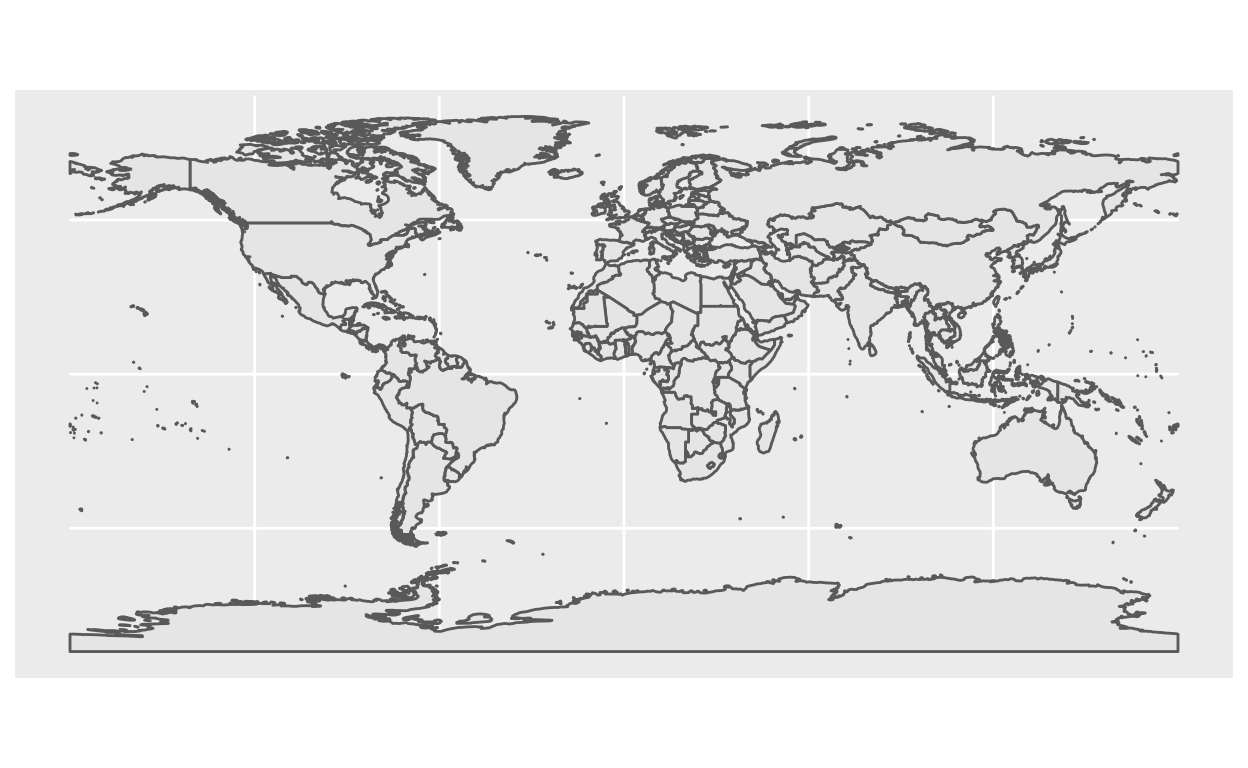#### Color and fill

``````ggplot(data = world) +
geom_sf(color = "black", fill = "lightgreen")
``````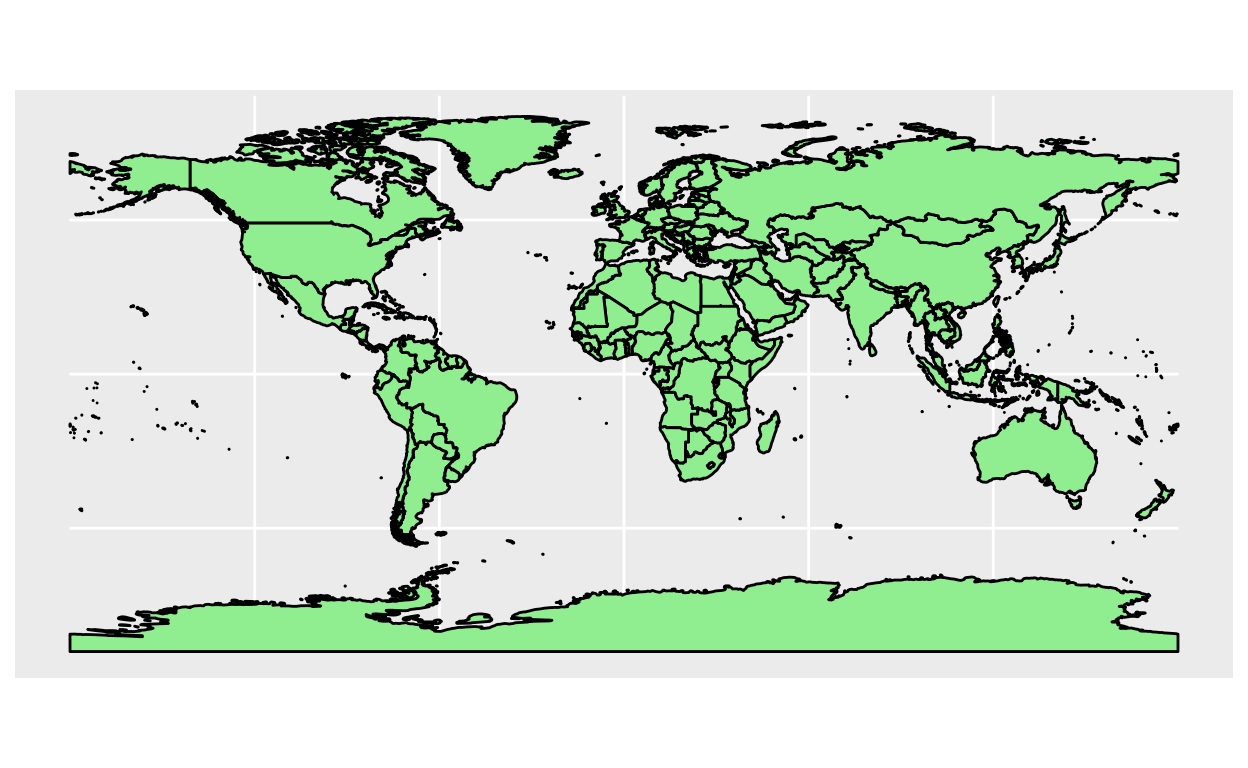### Coord_sf

``````coord_sf(xlim = NULL, ylim = NULL, expand = TRUE, crs = NULL, datum = sf::st_crs(4326),
label_graticule = waiver(), label_axes = waiver(), ndiscr = 100, default = FALSE, clip = "on")
``````

#### Crs

``````ggplot(data = world) +
geom_sf() +
coord_sf(crs = "+proj=laea +lat_0=52 +lon_0=10 +x_0=4321000 +y_0=3210000 +ellps=GRS80 +units=m +no_defs ")
``````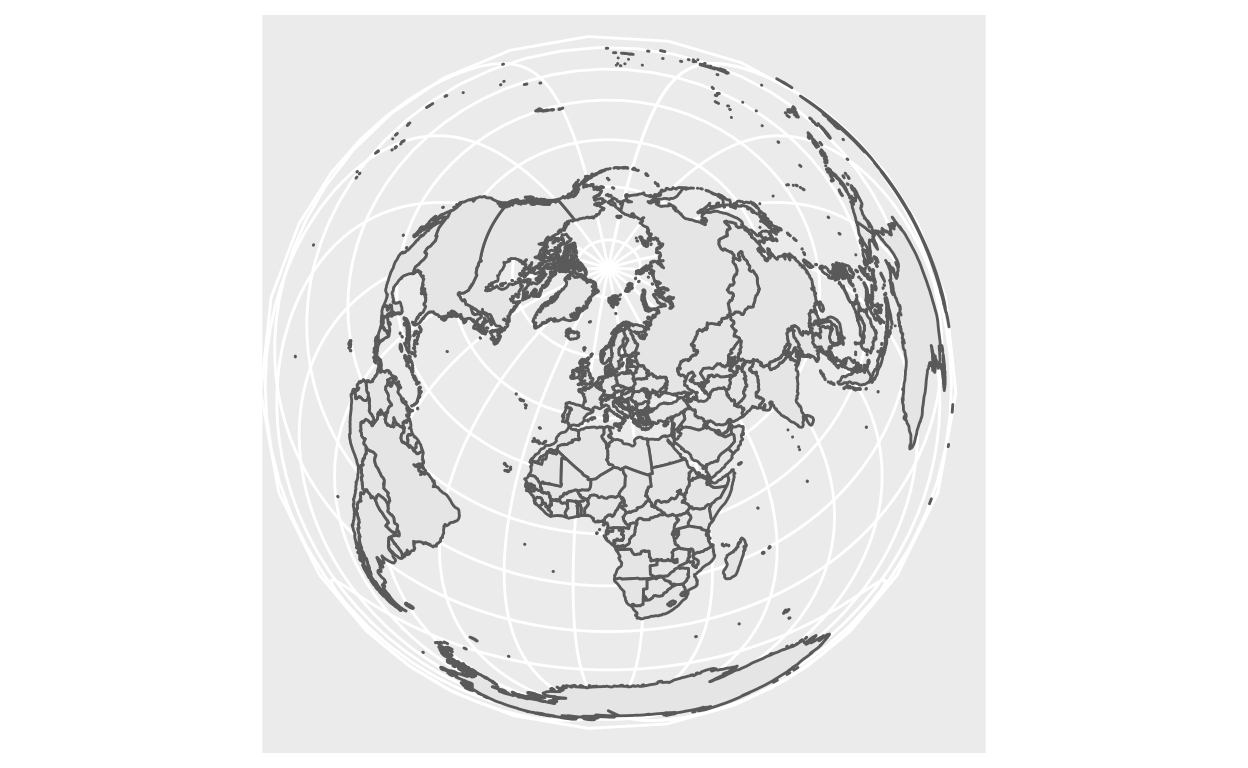#### Xlim and Ylim

``````ggplot(data = world) +
geom_sf() +
coord_sf(xlim = c(-102.15, -74.12), ylim = c(7.65, 33.97))
``````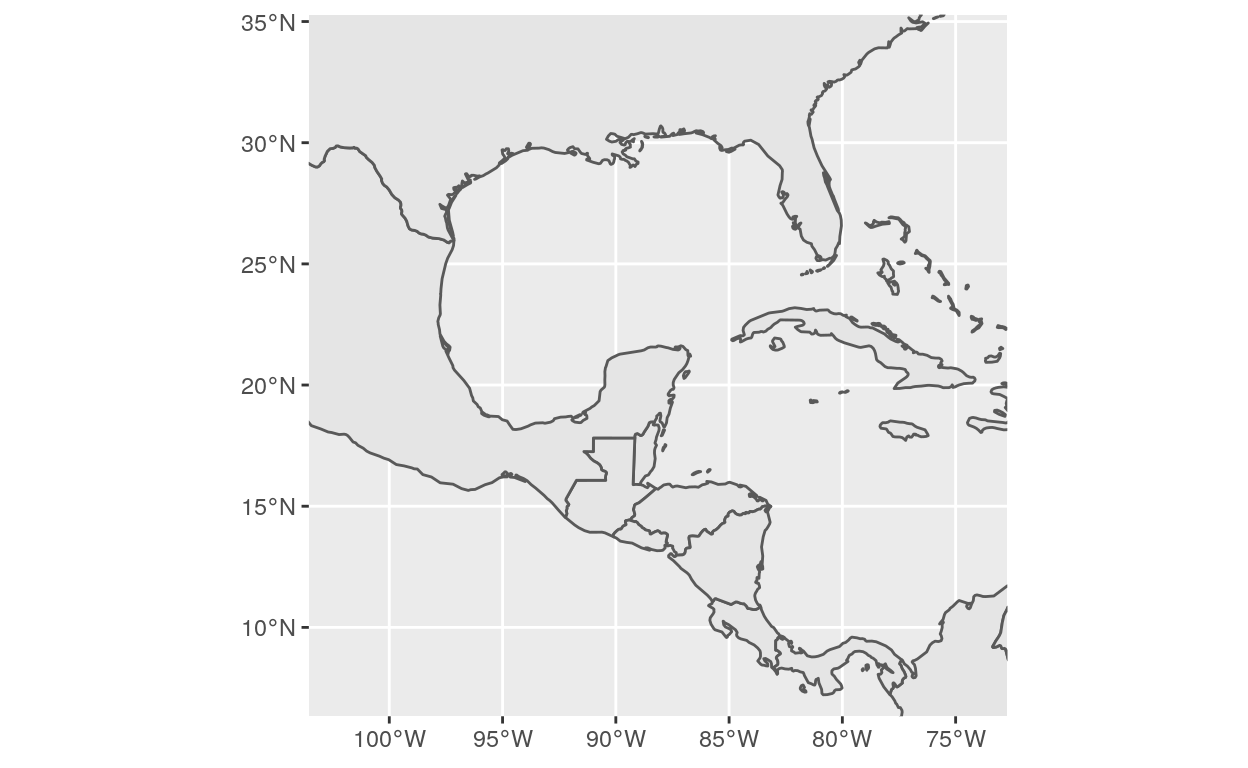### Geom_map

``````geom_map(mapping = NULL, data = NULL, stat = "identity", ..., map,
na.rm = FALSE, show.legend = NA, inherit.aes = TRUE)
``````

#### Map

``````# Use map_data() to load world data
world2 <- map_data('world')
# Show world2 data
``````
``````       long      lat group order region subregion
1 -69.89912 12.45200     1     1  Aruba      <NA>
2 -69.89571 12.42300     1     2  Aruba      <NA>
3 -69.94219 12.43853     1     3  Aruba      <NA>
4 -70.00415 12.50049     1     4  Aruba      <NA>
5 -70.06612 12.54697     1     5  Aruba      <NA>
6 -70.05088 12.59707     1     6  Aruba      <NA>``````
``````ggplot() +
geom_map(data=world2, map=world2, aes(x=long, y=lat, group=group, map_id=region))
``````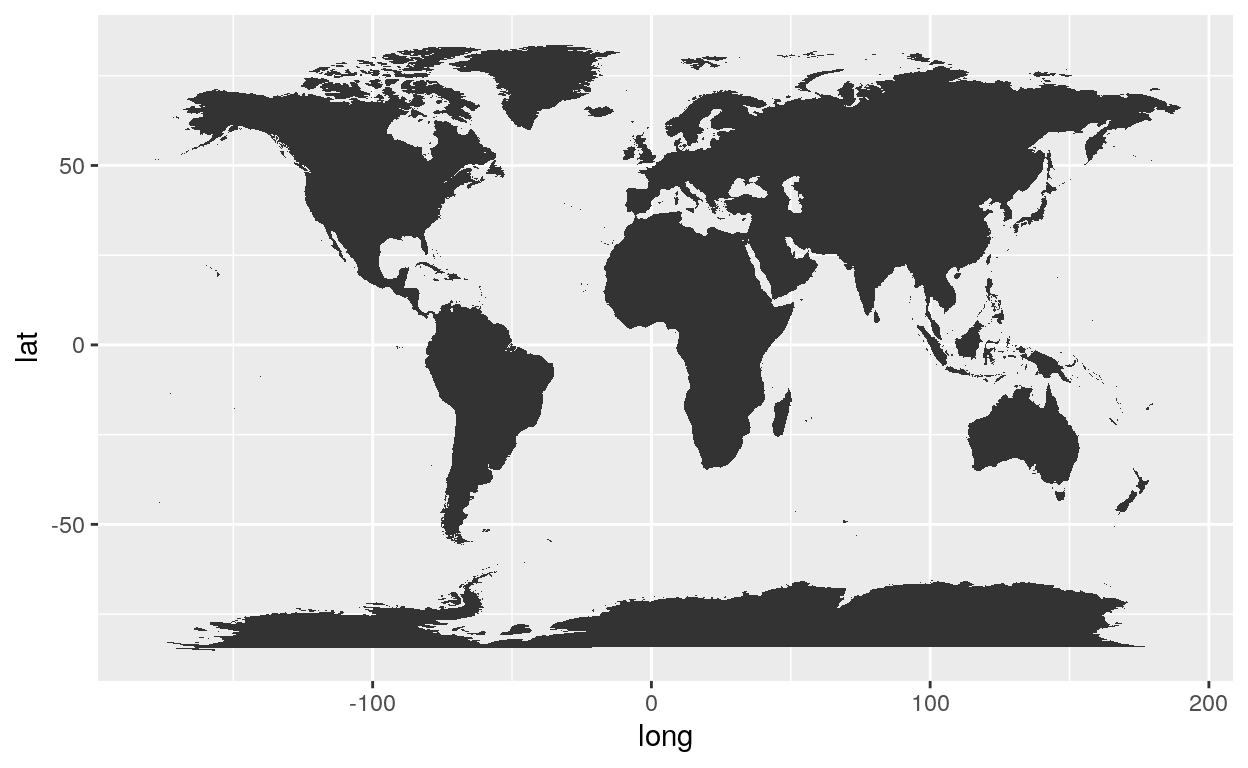### Coord_fixed

A fixed scale coordinate system forces a specified ratio between the physical representation of data units on the axes. The ratio represents the number of units on the y-axis equivalent to one unit on the x-axis. The default, ratio = 1, ensures that one unit on the x-axis is the same length as one unit on the y-axis. Ratios higher than one make units on the y axis longer than units on the x-axis, and vice versa.

``````coord_fixed(ratio = 1, xlim = NULL, ylim = NULL, expand = TRUE, clip = "on")
``````

#### Ratio

By default the Ration is equal to 1. When using another number the map shrinks.

``````ggplot() +
geom_map(data=world2, map=world2, aes(x=long, y=lat, group=group, map_id=region)) +
coord_fixed(1.9)
``````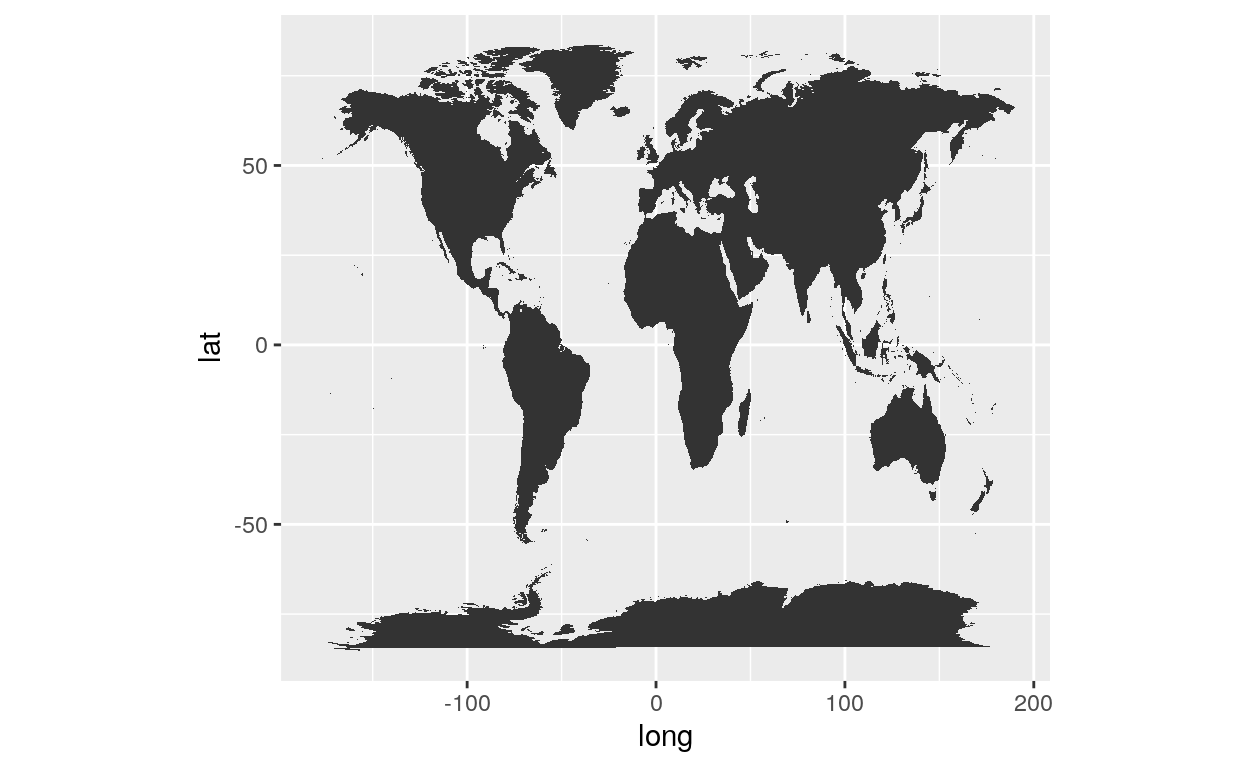#### Xlim and Ylim

You can cut the map by using the xlim and ylim option include in the `coord_fixed` function:

``````ggplot() +
geom_map(data=world2, map=world2, aes(x=long, y=lat, group=group, map_id=region)) +
coord_fixed(1.9, xlim = c(-160,175), ylim = c(-50,80))
``````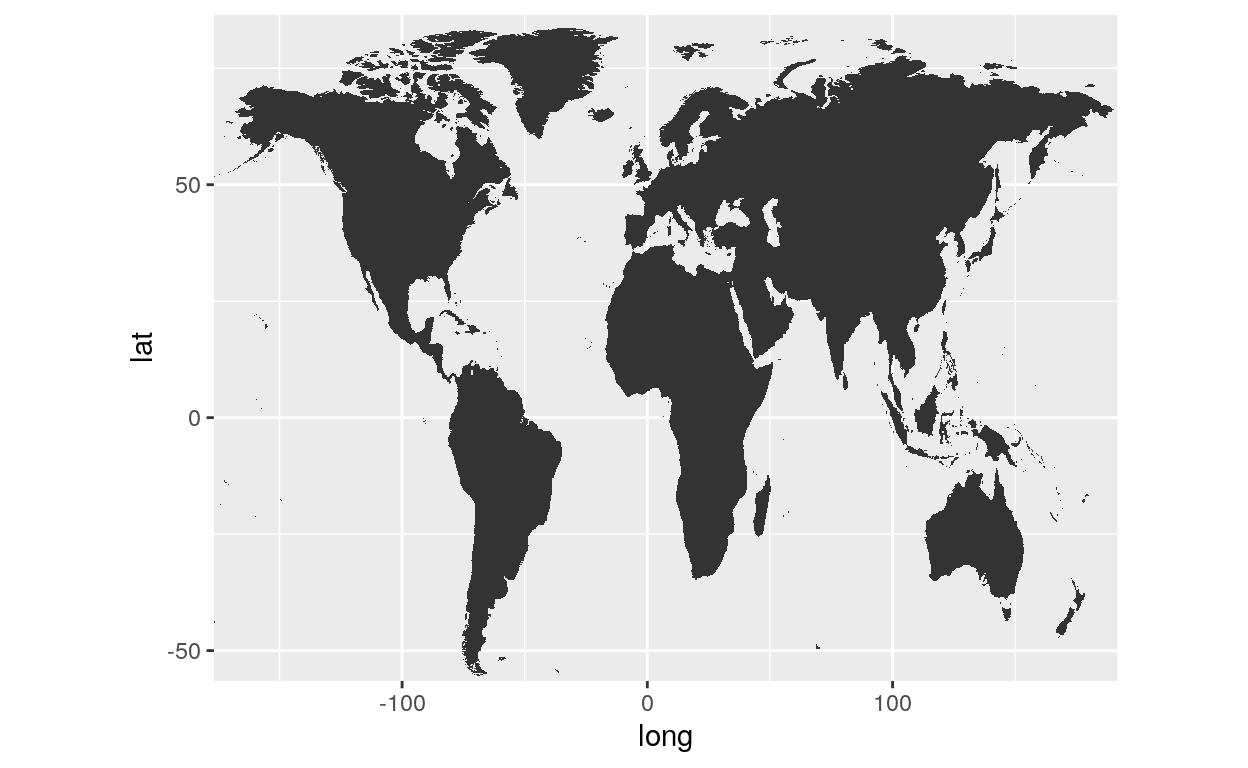### Geom_polygon

Polygons are very similar to paths (as drawn by geom_path()) except that the start and end points are connected and the inside is coloured by fill. The group aesthetic determines which cases are connected together into a polygon. From R 3.6 and onwards it is possible to draw polygons with holes by providing a subgroup aesthetic that differentiates the outer ring points from those describing holes in the polygon.

``````geom_polygon(mapping = NULL, data = NULL, stat = "identity", position = "identity", rule = "evenodd", ...,
na.rm = FALSE, show.legend = NA, inherit.aes = TRUE)
``````

#### Polygon

Let’s load US data with the function map_data().

``````library(maps)
library(ggmap)

usa <- map_data("usa")
``````
long lat group order region subregion
-101.4078 29.74224 1 1 main NA
-101.3906 29.74224 1 2 main NA
-101.3620 29.65056 1 3 main NA
-101.3505 29.63911 1 4 main NA
-101.3219 29.63338 1 5 main NA
-101.3047 29.64484 1 6 main NA
``````ggplot(usa, aes(x = long, y = lat, group = group)) +
geom_polygon()
``````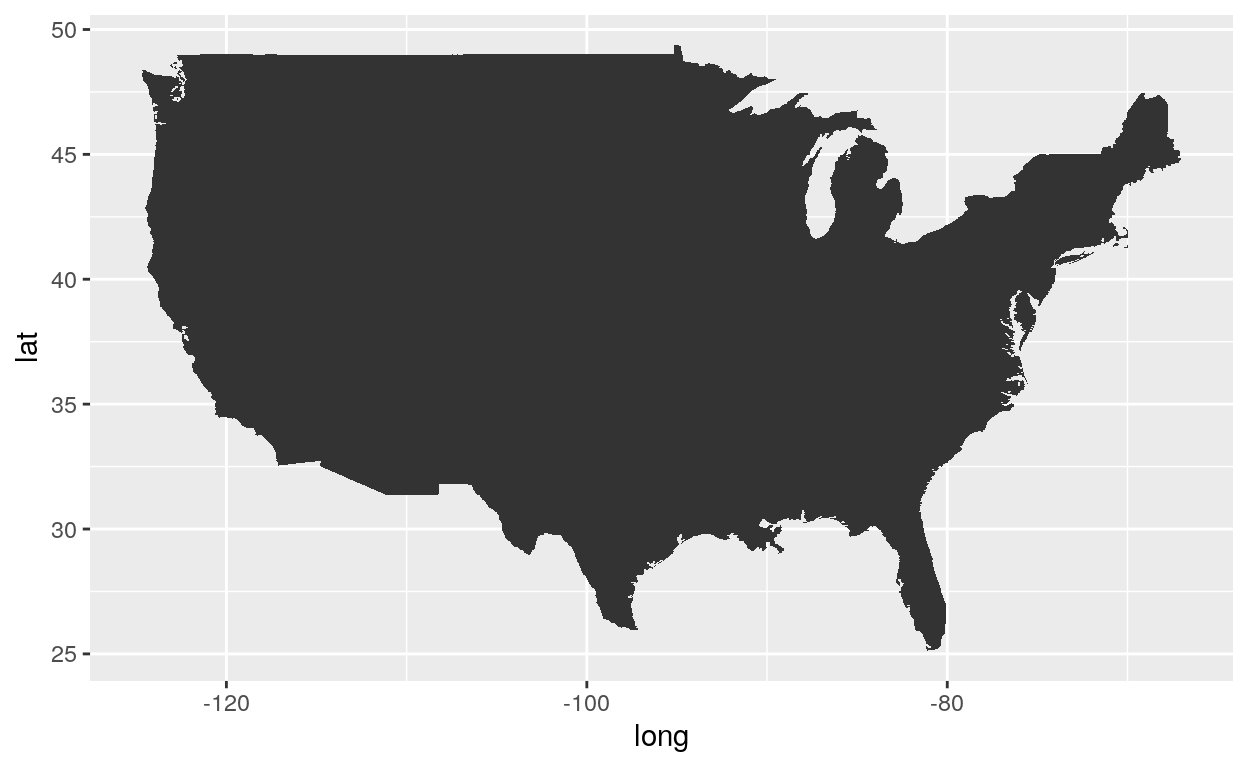### Coord_map

coord_map projects a portion of the earth, which is approximately spherical, onto a flat 2D plane using any projection defined by the mapproj package. Map projections do not, in general, preserve straight lines, so this requires considerable computation.

``````coord_map(projection = "mercator", ..., parameters = NULL, orientation = NULL,
xlim = NULL, ylim = NULL, clip = "on")
``````

#### Coord

``````ggplot(usa, aes(x = long, y = lat, group = group)) +
geom_polygon() +
coord_map()
``````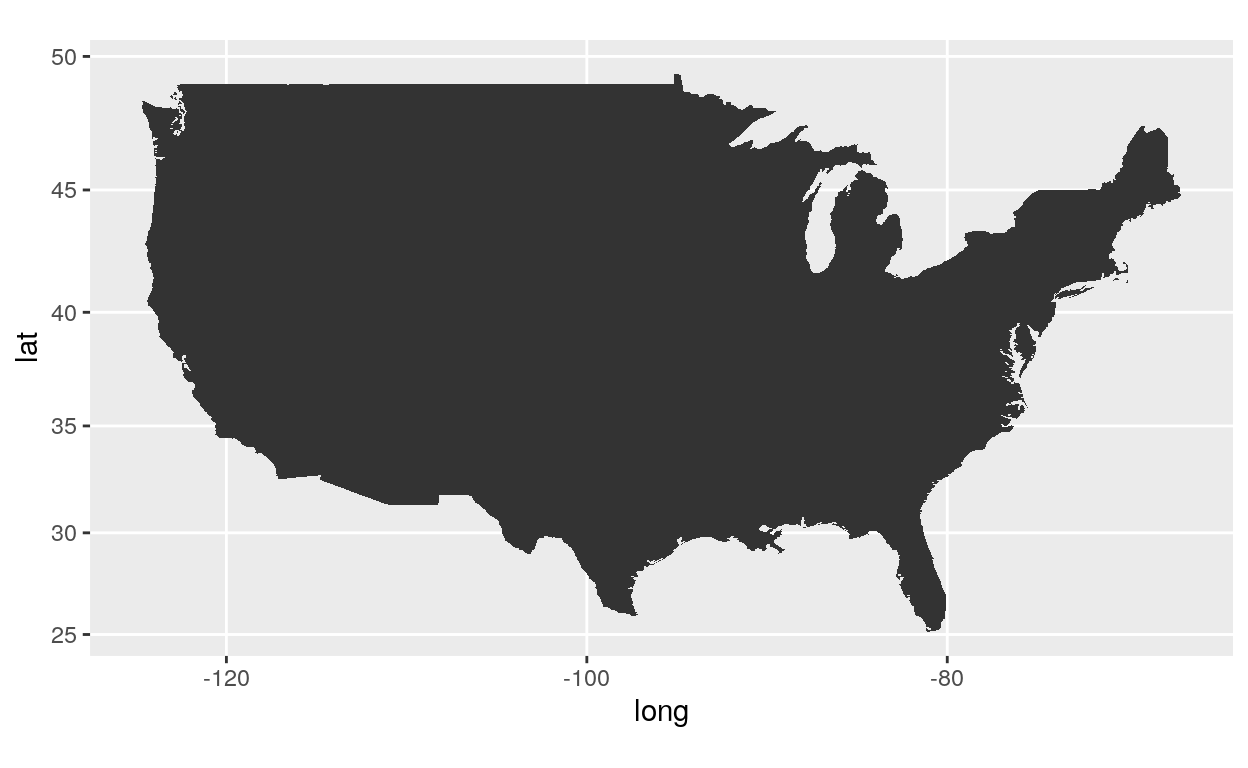#### Projection

``````ggplot(usa, aes(x = long, y = lat, group = group)) +
geom_polygon() +
coord_map("gilbert")
``````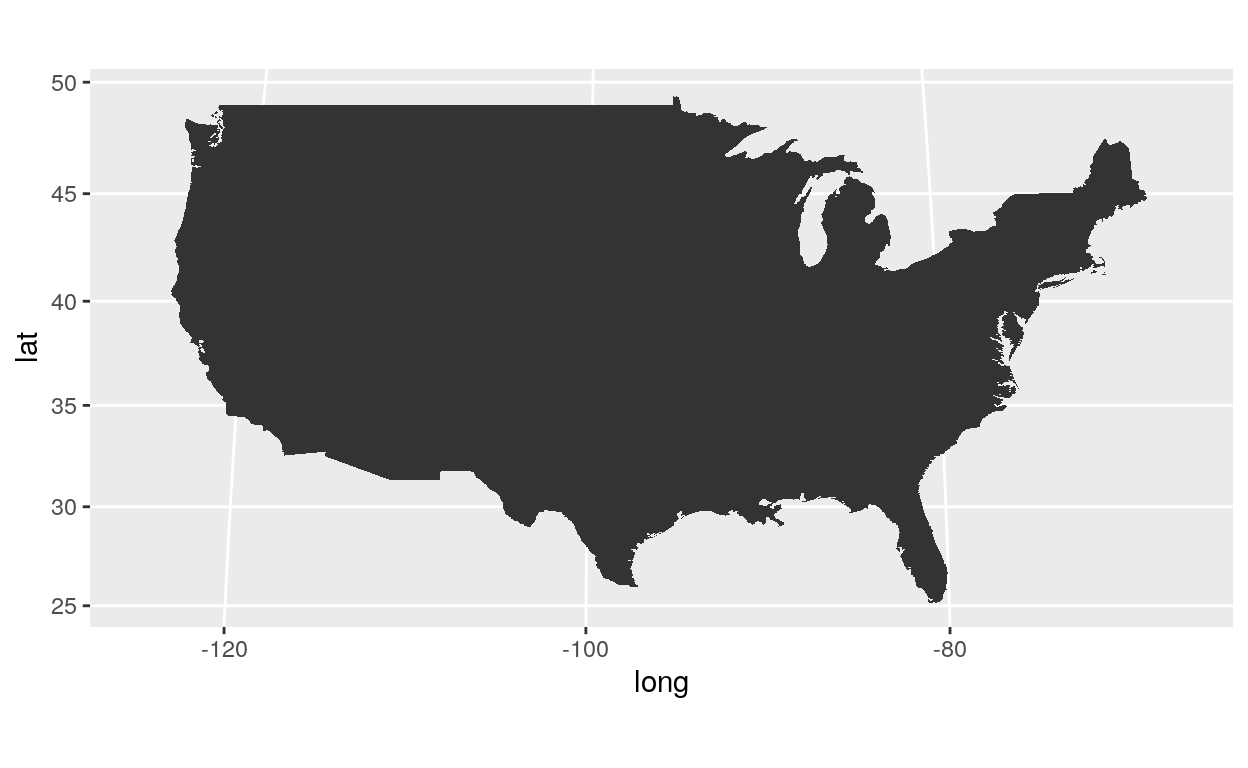``````ggplot(usa, aes(x = long, y = lat, group = group)) +
geom_polygon() +
coord_map("orthographic")
``````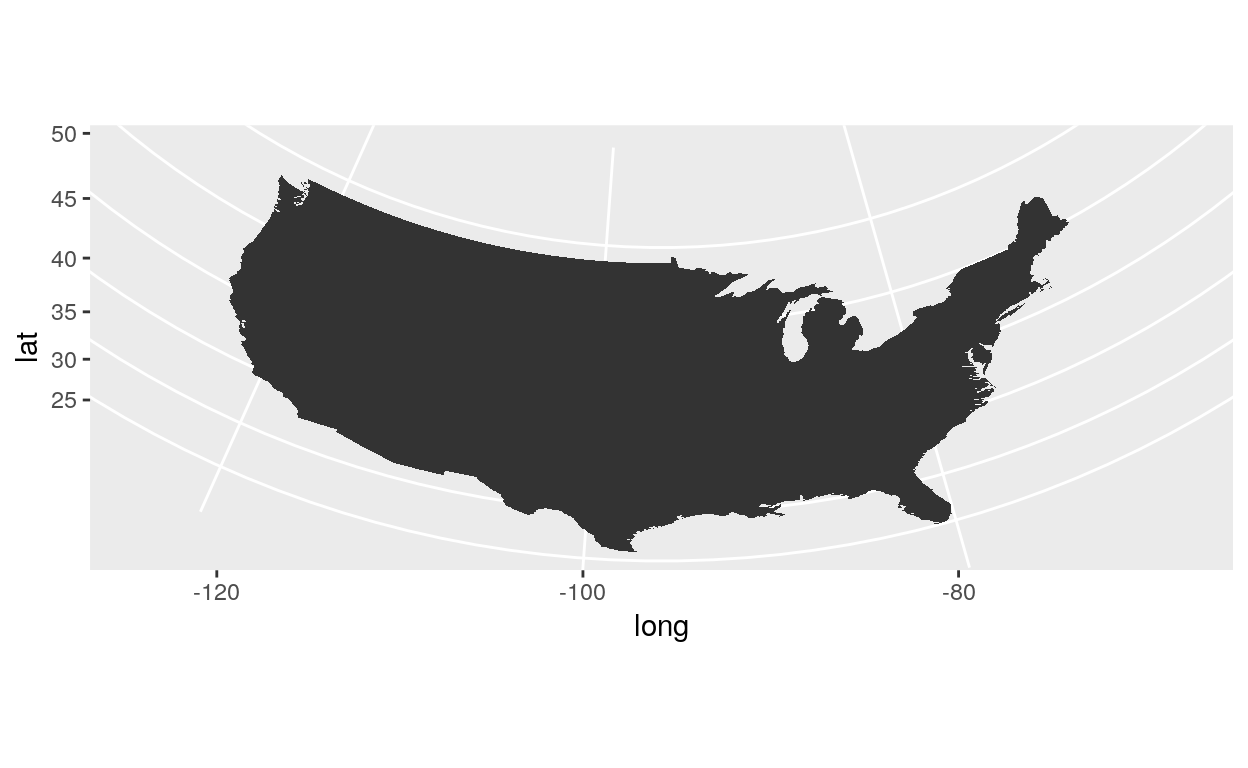``````ggplot(usa, aes(x = long, y = lat, group = group)) +
geom_polygon() +
coord_map("azequalarea")
``````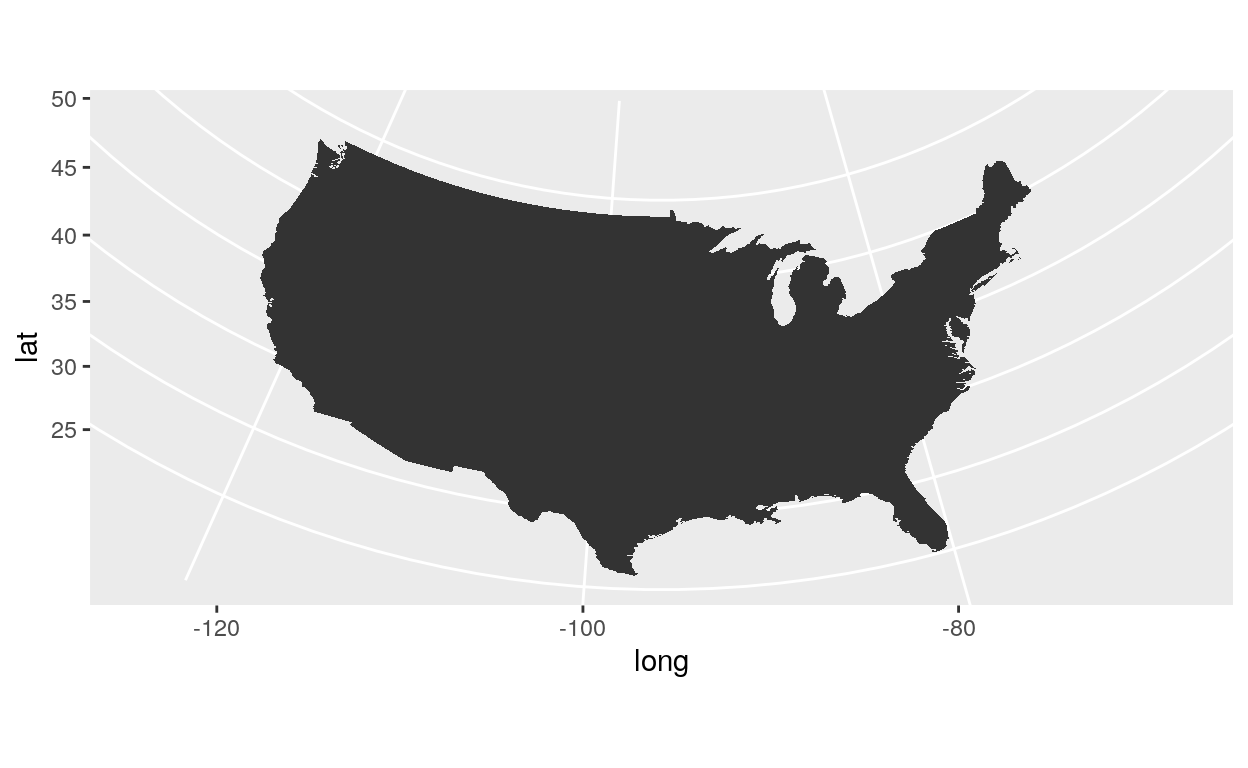``````ggplot(usa, aes(x = long, y = lat, group = group)) +
geom_polygon() +
coord_map("conic", lat0 = 30)
``````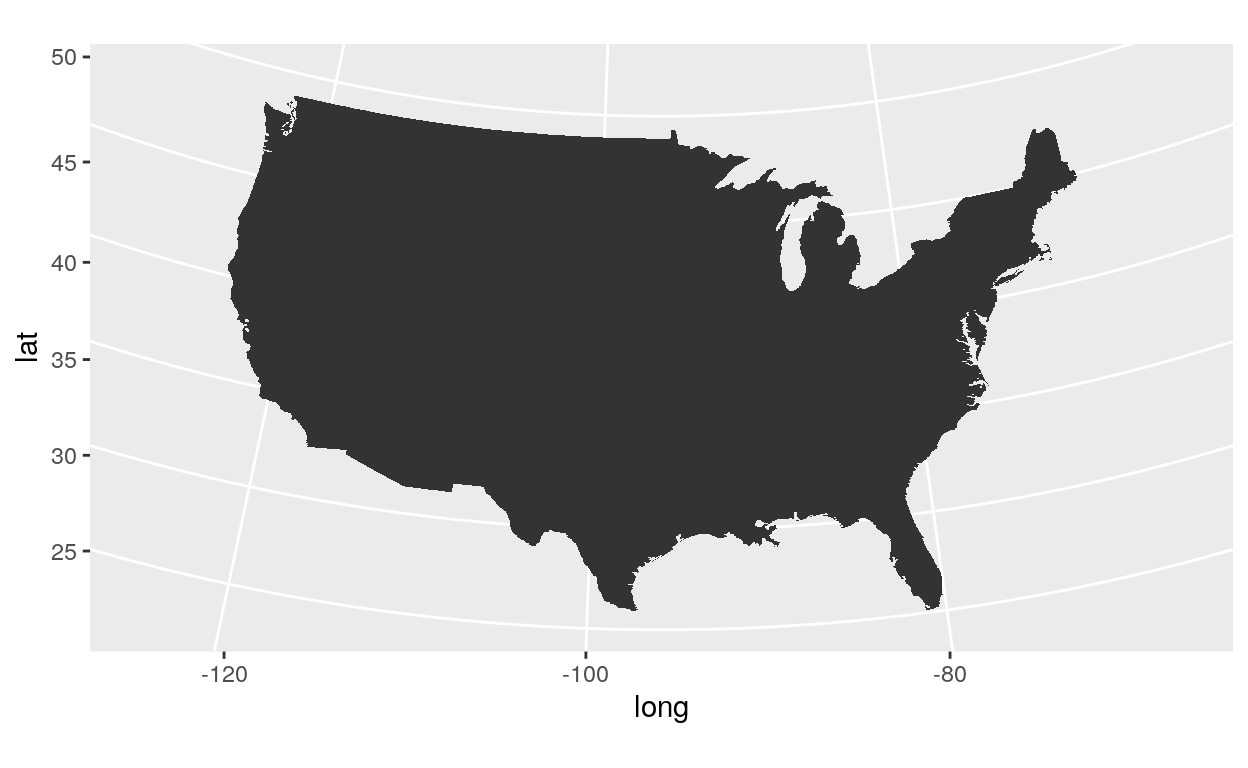Now, let’s load the US states data.

``````state <- map_data("state")
``````
long lat group order region subregion
-87.46201 30.38968 1 1 alabama NA
-87.48493 30.37249 1 2 alabama NA
-87.52503 30.37249 1 3 alabama NA
-87.53076 30.33239 1 4 alabama NA
-87.57087 30.32665 1 5 alabama NA
-87.58806 30.32665 1 6 alabama NA
``````ggplot(state, aes(x = long, y = lat, fill = region, group = group)) +
geom_polygon(col = "white") +
coord_map() +
theme(legend.position = "none")
``````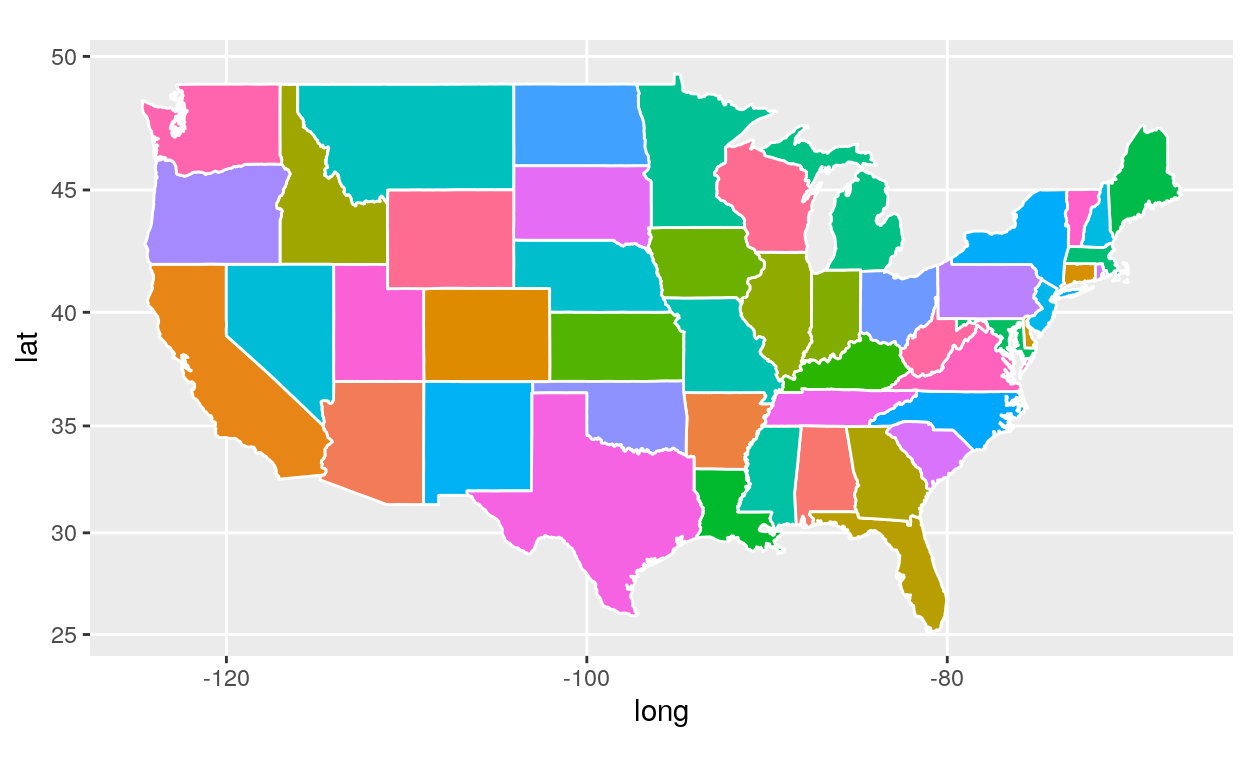### Points

For the following map we need data on cities. Let’s load them!

``````library(readr)
``````
City State Pop_est lat long
Eugene Oregon 163460 44.0567 -123.1162
Salem Oregon 164549 44.9237 -123.0231
Hillsboro Oregon 102347 45.5167 -122.9833
Santa Rosa California 174972 38.4468 -122.7061
Portland Oregon 632309 45.5370 -122.6500
Vancouver Washington 172860 45.6372 -122.5965
``````ggplot(data = usa, aes(x = long, y = lat, group = group)) +
geom_polygon() +
geom_point(data = cities, aes(group = State, size = Pop_est),
col = "red", shape = 19, alpha = 0.6) +
coord_map()
``````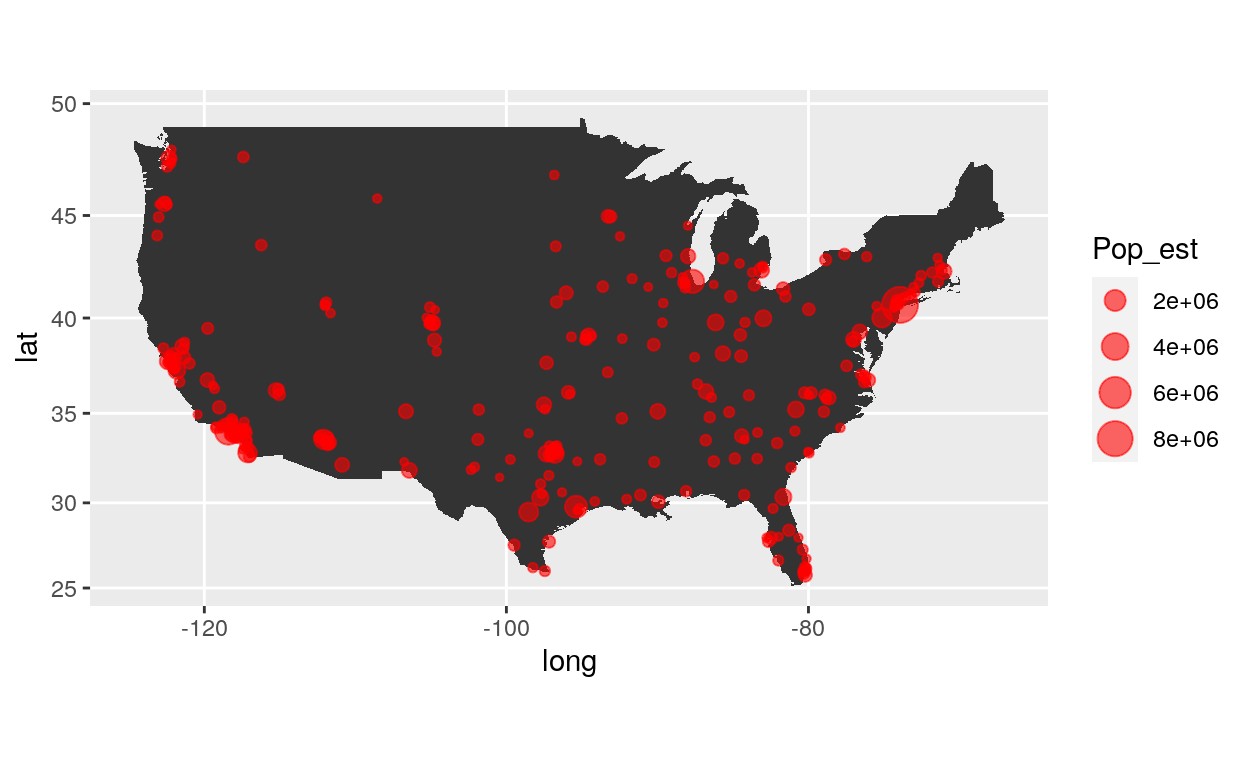We need to make some data wrangling on cities data.

First, we want the population by states.

``````pop <- aggregate(Pop_est ~ State, data = cities, sum)
``````

Second, we need to rename the column state by region.

``````names(pop)[names(pop) == "State"] <- "region"
``````

Third, we want to put in lowercase the column region

``````pop\$region <- stringr::str_to_lower(pop\$region)
``````

Next, we want to join the dataframe “state” and “pop” by the column “region” into a new dataframe called “state2”.

``````state2 <- dplyr::left_join(state, pop, by="region")
``````
long lat group order region subregion Pop_est
-87.46201 30.38968 1 1 alabama NA 797933
-87.48493 30.37249 1 2 alabama NA 797933
-87.52503 30.37249 1 3 alabama NA 797933
-87.53076 30.33239 1 4 alabama NA 797933
-87.57087 30.32665 1 5 alabama NA 797933
-87.58806 30.32665 1 6 alabama NA 797933

Now, let’s produce a map!

``````ggplot(state2, aes(x = long, y = lat, fill = Pop_est, group = group)) +
geom_polygon(col = "white") +
coord_map()
``````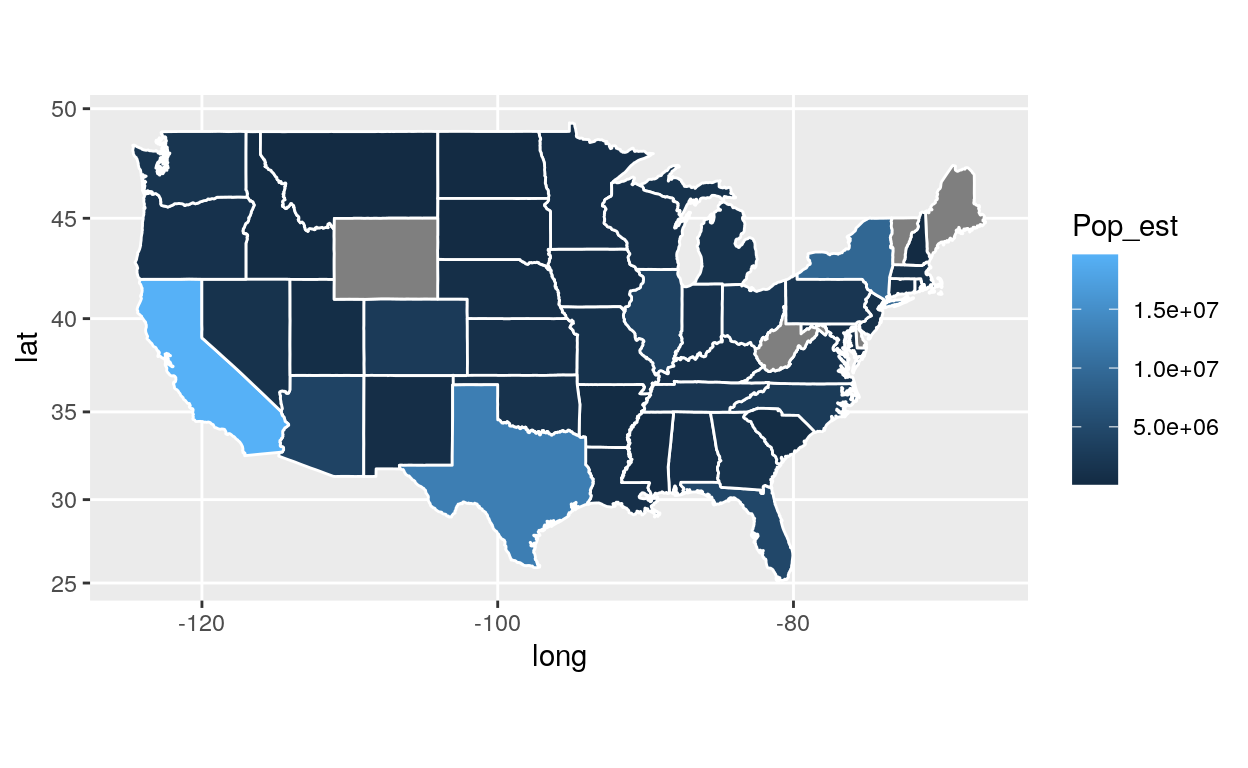### Citation

`Warin (2019, Aug. 5). Thierry Warin, PhD: [R Course] Data Visualization with R. Retrieved from https://warin.ca/posts/rcourse-datavisualizationwithr/`
```@misc{warin2019[r,